• Exam Center
• Ticket Center
• Flash Cards
• Straight Line Motion## Grade 12: Physics Worksheet on Projectile Motion

Looking to master projectile motion in your physics class? Check out our comprehensive worksheet with detailed solutions to help you understand the concepts and excel in your studies!

There is also a pdf  for worksheets with answers.

## A summary of projectile motion:

Any motion having the following conditions is called a projectile motion.     (i) Follows a parabolic path (trajectory).     (ii) Moves under the influence of gravity.

The projectile motion formulas applied to solve two-dimensional projectile motion problems are as follows \begin{gather*} x=(v_0\cos\theta)t+x_0\\\\y=-\frac 12 gt^2+(v_0\sin\theta)t+y_0\\\\ v_y=v_0\sin\theta-gt\\\\v_y^2-(v_0\sin\theta)^2=-2g(y-y_0)\end{gather*}

## Projectile motion problems and answers

Problem (1): A person kicks a ball with an initial velocity of 15 m/s at an angle of 37° above the horizontal (neglecting the air resistance). Find  (a) the total time the ball is in the air.  (b) the horizontal distance traveled by the ball

Solution : The initial step in answering any projectile motion questions is to establish a coordinate system and sketch the path of the projectile, including its initial and final positions and velocities.

By doing so, you will be able to solve the relevant projectile equations easily.

Hence, we choose the origin of the coordinate system to be at the throwing point, $x_0=0, y_0=0$.(a) Here, we are seeking the time it takes for the projectile to travel from the point of release to the ground.

In reality, projectiles have two independent motions: one in the horizontal direction with uniform motion at a constant velocity (i.e., $a_x=0$), and the other in the vertical direction under the effect of gravity (with $a_y=-g$).

The kinematic equations that describe the horizontal and vertical distances are as follows:  \begin{gather*} x=x_0+(\underbrace{v_0\cos \theta}_{v_{0x}})t \\ y=-\frac 12 gt^2+(\underbrace{v_0\sin \theta}_{v_{0y}})t+y_0\end{gather*} By substituting the coordinates of the initial and final points into the vertical equation, we can find the total time the ball is in the air.

Setting $y=0$ in the second equation (because the projectile lands at the same level as the throwing point.), we have: \begin{align*} y&=-\frac 12 gt^2+(v_0\sin \theta)t+y_0\\\\ 0&=-\frac 12 (9.8)t^2+(15)\sin 37^\circ\,t+0 \end{align*} By rearranging the above expression, we can obtain two solutions for $t$: \begin{gather*} t_1=0 \\\\ t_2=\frac{2\times 15\sin37^\circ}{9.8}=1.84\,{\rm s}\end{gather*} The first time represents the starting moment, and the second represents the total time the ball was in the air.

(b) As previously mentioned, projectile motion consists of two independent motions with different positions, velocities, and accelerations, each described by distinct kinematic equations.

The time it takes for the projectile to reach a specific point horizontally is equal to the time it takes to fall vertically to that point. Therefore, time is the common factor in both the horizontal and vertical motions of a projectile.

In this particular problem, the time calculated in part (a) can be used in the horizontal kinematic equation to determine the distance traveled.

Substituting the obtained time into the equation, we find that the projectile travels a distance of 22.08 meters. \begin{align*} x&=x_0+(v_0\cos \theta)t \\ &=0+(15)\cos 37^\circ\,(1.84) \\ &=22.08\quad {\rm m}\end{align*}

Be sure to check the following questions.

If you are getting ready for the AP Physics exam, these problems on the kinematics equation (or these AP Physics Kinematics Problems ) are probably also useful to you.

Problem (2): A ball is thrown into the air at an angle of 60° above the horizontal with an initial velocity of 40 m/s from a  50-m-high building. Find  (a) The time to reach the top of its path. (b) The maximum height the ball reached from the base of the building.

Solution : In the previous question, we found that the motion of a projectile consists of two distinct vertical and horizontal movements.

We learned about how to find distances in both directions using relevant kinematic equations.

There is also another set of kinematic equations that discuss the velocities in vertical and horizontal directions as follows \begin{gather*} v_x=v_{0x}=v_0\cos\theta \\ v_y=v_{0y}-gt=v_0\sin\theta-gt\end{gather*} As you can see, the horizontal component of the velocity, $v_x$, is constant throughout the motion, but the vertical component varies with time.

As an important note, keep in mind that in the problems about projectile motions, at the highest point of the trajectory, the vertical component of the velocity is always zero, i.e., $v_y=0$.

To solve the first part of the problem, specify two points as the initial and final points, then solve the relevant kinematic equations between those points.

Here, setting $v_y=0$ in the second equation and solving for the unknown time $t$, we have \begin{align*} v_y&=v_{0y}-gt \\\\ &=v_0\sin\theta-gt \\\\ 0&=(40)(\sin 60^\circ)-(9.8)(t) \\\\ \Rightarrow t&=\boxed{3.53\, {\rm s}}\end{align*} Thus, the time taken to reach the maximum height of the trajectory (path) is 3.53 seconds from the moment of the launch. We call this maximum time $t_{max}$.(b) Let the origin be at the throwing point, so $x_0=y_0=0$ in the kinematic equations. In this part, the vertical distance traveled to the maximum point is requested.

By substituting $t_{max}$ into the vertical distance projectile equation, we can find the maximum height as below \begin{align*} y-y_0&=-\frac 12 gt^2 +(v_0 \sin \theta)t\\ \\ y_{max}&=-\frac 12 (9.8)(3.53)^2+(40 \sin 60^\circ)(3.53)\\\\ &=61.22\quad {\rm m}\end{align*} Therefore, the maximum height at which the ball is reached from the base of the building is $H=50+y_{max}=111.22\quad {\rm m}$

For further reading about uniform motion along the horizontal direction, see  speed, velocity, and acceleration problems .

Problem (3): A person standing on the edge of a 50-m-high cliff throws a stone horizontally at a speed of 18 m/s.  (a) What is the initial position of the stone?  (b) What are the components of the initial velocity? (c) What are the $x$- and $y$-components of the velocity of the stone at any arbitrary time $t$? (d) How long will it take the stone to strike the bottom of the cliff? (e) With what angle and speed does the stone strike the ground below the cliff?Solution : As mentioned repeatedly, as a first step to solving a projectile motion problem, choose a relevant coordinate system.

(a) Usually, we place the origin of the coordinate system at the point where the projectile is thrown. In this case, the coordinate of the initial position is $x_0=0\, , \, y_0=0$.

If we had chosen the coordinate at the base of the cliff and placed the origin at that point, the position of the initial point would have been $x_0=0\, , \, y_0=50\,\rm m$.(b) The stone is thrown horizontally with $\theta=0$, so there is no vertical velocity component. Consequently, its initial speed components are $v_{0x}=18\,\rm m/s$ and $v_{0y}=0$.

(c) Remember that the velocity components of a projectile change over time according to the following formula. Substituting the given values into it, we get:  \begin{align*} v_x&=v_{0x}=18\,\rm m/s \\\\ v_y&=v_{0y}-gt\\&=-9.8t \end{align*}  (d) If we take the top of the cliff to be the origin of our coordinate system, with  $x_0=y_0=0$, the stone hits the ground $50\,\rm m$ below our chosen origin. Therefore, the coordinates of that point would be $x_0=?\, ,\, y=-50\,\rm m$.

Use the vertical displacement kinematic equation below, substitute the numerical values into that, and solve for time $t$ get \begin{gather*} y-y_0=-\frac 12 gt^2+v_{0y}t \\\\ -50-0=-\frac 12 (9.8)t^2-0 \\\\ \Rightarrow \quad \boxed{t=3.2\,\rm s} \end{gather*}  (e) First, find the velocity components just before the stone hits the ground. We know that it takes about $3.2\,\rm s$ for the stone to reach the bottom of the cliff. Thus, put this time value into the formulas of velocity components at any time $t$. \begin{align*} v_x&=v_{0x}=18\,\rm m/s \\\\ v_y&=v_{0y}-gt\\&=-9.8\times 3.2 \\&=-31.36\,\rm m/s \end{align*} Notice that the negative sign here indicates that the vertical velocity component just before hitting the ground points downward, as expected.

Recall from the section on  vector practice problems that when we have the components of a vector, we can find its magnitude using the Pythagorean theorem. This gives us: \begin{align*} v&=\sqrt{v_x^2+v_y^2} \\\\ &=\sqrt{18^2+(-31.36)^2} \\ &=\boxed{36.15\,\rm m/s} \end{align*} We can also find the angle of impact with the ground using the velocity components: \begin{align*} \tan\theta&=\frac{v_y}{v_x} \\\\ &=\frac{-31.36}{18} \\\\ &=-1.74 \end{align*} Taking the inverse tangent of both sides gives: $\theta=\tan^{-1}(-1.74)=\boxed{-60.1^\circ}$ Therefore, the stone hit the ground at an angle of about $60^\circ$ with a speed of $36.15\,\rm m/s$. The negative angle indicates that it is below horizontal, which is to be expected.

Problem (4): A book slides off a frictionless tabletop with a constant speed of 1.1 m/s, and 0.35 seconds later strikes the floor.  (a) How high is the tabletop from the floor? (b) What is the horizontal distance to that point where the book strikes the floor? (c) How fast and at what angle does the book strike the floor?

Solution : The total time the book is in the air is $t=0.35\,\rm s$, and the initial speed with which the book leaves the table horizontally is $v_{0x}=1.1\,\rm m/s$, and $v_{0y}=0$.

Contrary to the earlier question, in this case, for practice, we place the origin at the bottom of the tabletop. Thus, the coordinates of the initial position would be $x_0=0\, ,\, y_0=h=?$ and the coordinates of the landing point would be $x=? \, , \, y=0$.

(a) If we substitute the given values into the vertical displacement kinematic equation, we will have \begin{gather*} y-y_0=-\frac 12 gt^2+v_{0y}t \\\\ 0-h=-\frac 12 (9.8)(1.1)^2+0 \\\\ \Rightarrow \quad \boxed{h=5.93\,\rm m} \end{gather*} Thus, the table is 3-m-high.

(b)  Remember that projectile motion involves two motions that are uniformly accelerated in the horizontal and vertical directions.  The time taken by the projectile in the vertical direction is equal to the horizontal time to the landing point.

Between the start and final points in the horizontal direction of the projectile path, we can write $\Delta x=v_{0x}t$. Substituting the given values into is get \begin{gather*} x-0=1.1\times (0.35) \\ \Rightarrow \quad \boxed{x=0.385\,\rm m} \end{gather*} where $\Delta x=x-x_0$. Therefore, the book strikes the floor about $39\,\rm cm$ ahead of the base of the table.

(c) This part is straightforward. Substitute the given numerical values into the velocity vector component formulas as below to find the velocity components just before the book strikes the floor. \begin{align*} v_x&=v_{0x}=1.1\,\rm m/s \\\\ v_y&=v_{0y}-gt \\\\ &=0-(9.8)(0.35) \\&=-3.43\,\rm m/s \end{align*} The square root of the sum of the velocity components squared gives the velocity of the book at the instant of hitting \begin{align*} v&=\sqrt{v_x^2+v_y^2} \\\\ &=\sqrt{1.1^2+(-3.43)^2} \\\\ &=\boxed{3.6\,\rm m/s} \end{align*}  and angle of impact with the ground is found as below \begin{align*} \theta&=\tan^{-1}\left(\frac{v_y}{v_x}\right) \\\\ &=\tan^{-1}\left(\frac{-3.43}{1.1}\right) \\\\ &=\tan^{-1}(-3.11) \\\\&=\boxed{-72.17^\circ} \end{align*} Overall, the book hit the ground at an angle of about $72^\circ$ with a speed of nearly $3.6\,\rm m/s$.

Problem (5): A cannonball is fired from a cliff with a speed of 800 m/s at an angle of 30° below the horizontal. How long will it take to reach 150 m below the firing point?

Solution: First, choose the origin to be at the firing point, so $x_0=y_0=0$. Now, list the known values as follows      (i) Projectile's initial velocity = $800\,{\rm m/s}$      (ii) Angle of projectile: $-30^\circ$, the negative sign is because the throw is below the horizontal.     (iii) y-coordinate of the final point, 150 m below the origin, $y=-150\,{\rm m}$.       In this problem, the time it takes for the cannonball to reach 100 m below the starting point is required.

Since the displacement to that point is known, we apply the vertical displacement projectile formula to find the needed time as below \begin{align*} y-y_0&=-\frac 12 gt^2+(v_0\sin\theta)t\\\\-150&=-\frac 12 (9.8)t^2+(800)\sin(-30^\circ)t\\\\ \Rightarrow & \ 4.9t^2+400t-150=0\end{align*} The above quadratic equation has two solutions, $t_1=0.37\,{\rm s}$ and $t_2=-82\,{\rm s}$. It is obvious that the second time is not acceptable.

Therefore, the cannonball takes 0.37 seconds to reach 150 meters below the firing point.

Problem (6): Someone throws a stone into the air from ground level. The horizontal component of velocity is 25 m/s and it takes 3 seconds for the stone to come back to the same height as before. Find  (a) The range of the stone. (b) The initial vertical component of velocity (c) The angle of projection.

Solution: The known values are      (i) The initial horizontal component of velocity, $v_{0x}=25\,{\rm m/s}$.      (ii) The time between the initial and final points, which are at the same level, $t=3\,{\rm s}$.

(a)  The range of projectile motion is defined as the horizontal distance between the launch point and impact at the same elevation.

Because the horizontal motion in projectiles is a motion with constant velocity, the distance traveled in this direction is obtained as $x=v_{0x}t$, where $v_{0x}$ is the initial component of the velocity.

If you put the total time the projectile is in the air into this formula, you get the range of the projectile.

In this problem, the stone is thrown from the ground level and, after 3 seconds, reaches the same height. Thus, this is the total time of the projectile.

Hence, the range of the stone is found as below \begin{align*} x&=v_{0x}t\\&=(25)(3)\\&=75\,{\rm m}\end{align*}

(b)  The initial vertical component of the projectile's velocity appears in two equations, $v_y=v_{0y}-gt$ and $y-y_0=-\frac 12 gt^2+v_{0y}t$.

Using the second formula is more straightforward because the stone reaches the same height, so its vertical displacement between the initial and final points is zero, i.e., $y-y_0=0$. Setting this into the vertical distance projectile equation, we get \begin{align*} y-y_0&=-\frac 12 gt^2+v_{0y}t\\\\ 0&=-\frac 12 (9.8)(3)^2+v_{0y}(3) \\\\ \Rightarrow v_{0y}&=14.7\quad{\rm m/s}\end{align*} To use the first formula, we need some extra facts about projectile motion in the absence of air resistance, as below      (i) The vertical velocity is zero at the highest point of the path of the projectile, i.e., $v_y=0$.     (ii) If the projectile lands at the same elevation from which it was launched, then the time it takes to reach the highest point of the trajectory is half the total time between the initial and final points.      The second note, in the absence of air resistance, is only valid.

In this problem, the total flight time is 3 s because air resistance is negligible, so 1.5 seconds are needed for the stone to reach the maximum height of its path.

Therefore, using the second equation, we can find $v_{0y}$ as below \begin{align*} v_y&=v_{0y}-gt\\0&=v_{0y}-(9.8)(1.5) \\\Rightarrow v_{0y}&=14.7\quad {\rm m/s}\end{align*}  (c) The projection angle is the angle at which the projectile is thrown into the air and performs a two-dimensional motion.

Once the components of the initial velocity are available, using trigonometry, we can find the angle of projection as below \begin{align*} \theta&=\tan^{-1}\left(\frac{v_{0y}}{v_{0x}}\right)\\\\&=\tan^{-1}\left(\frac{14.7}{25}\right)\\\\&=+30.45^\circ\end{align*} Therefore, the stone is thrown into the air at an angle of about $30^\circ$ above the horizontal.

Problem (7): A ball is thrown at an angle of 60° with an initial speed of 200 m/s. (Neglect the air resistance.) (a) How long is the ball in the air? (b) Find the maximum horizontal distance traveled by the ball. (c) What is the maximum height reached by the ball?

Solution: We choose the origin to be the ball's initial position so that $x_0=y_0=0$. The given data is      (i) The projection angle: $60^\circ$.     (ii) Initial speed : $v_0=200\,{\rm m/s}$.

(a) The initial and final points of the ball are at the same level, i.e., $y-y_0=0$.

Thus, the total time the ball is in the air is found by setting $y=0$ in the projectile equation $y=-\frac 12 gt^2+v_{0y}t$ and solving for time $t$ as below \begin{align*} y&=-\frac 12 gt^2+(v_0\sin\theta)t\\\\0&=-\frac 12 (9.8)t^2+(200)(\sin 60^\circ)t\\\\\Rightarrow \quad & \boxed{(-4.9t+100\sqrt{3})t=0} \end{align*} The above expression has two solutions for $t$. One is the initial time, $t_1=0$, and the other is computed as $t_2=35.4\,{\rm s}$.

Hence, the ball has been in the air for about 35 s.

(b) The horizontal distance is called the range of the projectile. By inserting the above time (total flight time) into the horizontal distance projectile equation $x=v_{0x}t$, we can find the desired distance traveled. \begin{align*} x&=(v_0\cos\theta)t\\&=(200)(\cos 60^\circ)(35.4)\\&=3540 \quad {\rm m}\end{align*} Therefore, the ball hits the ground 3540 meters away from the throwing point.

(c) Using the projectile equation $v_y^2-v_{0y}^2=-2g(y-y_0)$, setting $v_y=0$ at the highest point of the path, and solving for the vertical distance $y$, the maximum height is found as follows \begin{align*} v_y^2-v_{0y}^2&=-2g(y-y_0)\\0-(200\sin 60^\circ)^2&=-2(9.8)y\\\Rightarrow y&=1531\quad {\rm m}\end{align*} Another method: As mentioned above, the ball hits the ground at the same level as before, so by knowing the total flight time and halving it, we can find the time it takes the ball to reach the highest point of its trajectory.

Therefore, by setting the half of the total flight time in the following projectile kinematic formula and solving for $y$, we can find the maximum height as \begin{align*} y-y_0&=-\frac 12 gt^2+(v_0\sin\theta)t\\\\y&=-\frac 12 (9.8)(17.7)^2+(200\sin60^\circ)(17.7)\\\\&=1531\quad {\rm m}\end{align*} Hence, the ball reaches 1531 meters above the launch point.

Problem (8): What are the horizontal range and maximum height of a bullet fired with a speed of 20 m/s at 30° above the horizontal?

Solution : first, find the total flight time, then insert it into the horizontal displacement projectile equation $x=v_{0x}t$ to find the range of the bullet.

Because the bullet lands at the same level as the original, its vertical displacement is zero, $y-y_0=0$, in the following projectile formula we can find the total flight time \begin{align*} y-y_0&=-\frac 12 gt^2+(v_0\sin\theta)t\\\\0&=-\frac 12 (9.8)t^2+(20)(\sin30^\circ)t\\\\ \Rightarrow & (-4.9t+10)t=0\end{align*} Solving for time $t$ gives two solutions: one is the initial time $t_1=0$, and the other is $t_2=1.02\,{\rm s}$. Thus the total time of flight is 2.04 s.

Therefore, the maximum horizontal distance traveled by the bullet, which is defined as the range of the projectile, is calculated as \begin{align*} x&=(v_0\cos\theta)t\\&=(20\cos 30^\circ)(2.04)\\&=35.3\quad {\rm m}\end{align*} Hence, the bullet lands about 17 m away from the launch point.

Because the air resistance is negligible and the bullet lands at the same height as the original, the time it takes to reach the highest point of its path is always half the total flight time.

On the other hand, recall that the vertical component of velocity at the maximum height is always zero, i.e., $v_y=0$. By inserting these two notes into the following projectile equation, we have \begin{align*} y&=-\frac 12 gt^2+(v_0\sin\theta)t\\\\&=-\frac 12 (9.8)(1.02)^2+(20\sin 30^\circ)(1.02)\\\\&=5.1\quad {\rm m}\end{align*} We could also use the kinematic equation $v_y^2-v_{0y}^2=-2g(y-y_0)$, to find the maximum height as below \begin{align*} v_y^2-(v_0 \sin \theta)^2 &=-2g(y-y_0) \\ 0-(20\sin 30^\circ)^2 &=-2(9.8)H\\ \Rightarrow H&= 5.1\quad {\rm m} \end{align*} I think the second method is much simpler.

Problem (9): A projectile is fired horizontally at a speed of 8 m/s from an 80-m-high cliff. Find  (a) The velocity just before the projectile hits the ground.  (b) The angle of impact.

Solution : In this problem, the angle of the projectile is zero because it is fired horizontally.

The velocity at each point of a projectile trajectory (path) is obtained by the following formula: $v=\sqrt{v_x^2+v_y^2}$ where $v_x$ and $v_y$ are the horizontal and vertical components of the projectile's velocity at any instant of time.

(a) Recall that the horizontal component of the projectile's velocity is always constant and, for this problem, is found as \begin{align*} v_x&=v_0\cos\theta\\&=8\times \cos 0^\circ\\&=8\quad {\rm m/s}\end{align*} To find the vertical component of the projectile velocity at any moment, $v_y=v_0\sin\theta-gt$, we should find the time taken to reach that point.

In this problem, that point is located just before striking the ground, whose coordinates are $y=-80\,{\rm m}, x=?$.

Because it is below the origin, which is assumed to be at the firing point, we inserted a minus sign.

Because displacement in the vertical direction is known, we can use the projectile formula for vertical distance.

By setting $y=-80$ into it and solving for the time $t$ needed the projectile reaches the ground, we get \begin{align*} y&=-\frac 12 gt^2+(v_0\sin\theta)t\\\\-80&=-\frac 12 (9.8)t^2+(8\times \sin 0^\circ)t\\\\\Rightarrow t&=\sqrt{\frac{2(80)}{9.8}}\\\\&=2.86\quad {\rm s}\end{align*} Now insert this time into the $y$-component of the projectile's velocity to find $v_y$ just before hitting the ground \begin{align*} v_y&=v_0\sin\theta-gt\\ &=8\sin 0^\circ-(9.8)(2.86)\\&=-28\quad {\rm m/s}\end{align*} Now that both components of the velocity are available, we can compute its magnitude as below \begin{align*} v&=\sqrt{v_x^2+v_y^2}\\\\&=\sqrt{8^2+(-28)^2}\\\\&=29.1\quad {\rm m/s}\end{align*} Therefore, the projectile hits the ground at a speed of 29.1 m/s.

(b) At any instant of time, the velocity of the projectile makes some angle with the horizontal, whose magnitude is obtained as the follows: $\alpha=\tan^{-1}\left(\frac{v_y}{v_x}\right)$ Substituting the above values into this formula, we get $\alpha =\tan^{-1}\left(\frac{-28}{8}\right)=-74^\circ$ Therefore, the projectile hits the ground at an angle of 74° below the horizontal.

Problem (10): From a cliff 100 m high, a ball is kicked at $30^\circ$ above the horizontal with a speed of $20\,{\rm m/s}$. How far from the base of the cliff did the ball hit the ground? (Assume $g=10\,{\rm m/s^2}$).

Solution: Again, similar to any projectile motion problem, we first select a coordinate system and then draw the path of the projectile as shown in the figure below,

We choose the origin to be at the kicking point above the cliff, setting $x_0=y_0=0$ in the kinematic equations.

The coordinate of the hitting point to the ground is $y=-100\,{\rm m}, x = ?$. A negative is inserted because the final point is below the origin.

Now, we find the common quantity in projectile motions—that is, the time between the initial and final points, called total flight time.

To find the total time the ball was in the air, we can use the vertical equation and solve for the unknown $t$ as follows \begin{align*} y&=-\frac 12 gt^2 +(v_0\sin \theta)t \\\\ -100&=-\frac 12 (10) t^2+(20\sin 30^\circ)t\\\\&\Rightarrow \quad \boxed{t^2-2t-20=0} \end{align*} The solutions of a quadratic equation $at^2+bt+c=0$ are found by the formula below $t=\frac{-b\pm\sqrt{b^2-4ac}}{2a}$ By matching the above constant coefficients with the standard quadratic equation, we can find the total time as below $t=\frac{-(-2)\pm\sqrt{(-2)^2-4(1)(-20)}}{2(1)}$ After simplifying, two solutions are obtained, $t_1=5.6\,{\rm s}$ and $t_2=-3.6\,{\rm s}$.

It is obvious that time cannot be negative in physics, so the acceptable answer is $t_1$.

It is the time it takes the ball to travel in the vertical direction. On the other hand, it is also the time it takes the ball to travel the horizontal distance between kicking and hitting points.

We insert this time into the horizontal equation to find the horizontal distance traveled, known as the projectile's range. \begin{align*} x&=(v_0\cos \theta)t\\ &=(20)(\cos 30^\circ)(5.6)\\&=97\quad {\rm m}\end{align*}

Problem (11): A cannonball is fired from the top of a $200\,{\rm m}$ cliff with a speed of $60\,{\rm m/s}$ at an angle of $60^\circ$ above the horizontal. Where does the ball land closest to which of the following choices? (Neglect air resistance.) (a) 90m        (b) 402 m        (c) 151 m     (d) 200 mSolution : The horizontal distance from the point where the cannonball fired is wanted, $x=?$. The appropriate kinematic equation for this is $x=(v_0\cos\theta)t$ The only thing we need is the total time the ball is in the air before landing.

If we take the firing point as the origin, then the cannonball lands $200\,{\rm m}$ below the origin. So, the ball's vertical displacement is $\Delta y=-200\,{\rm m}$. Set this in the vertical displacement equation, $\Delta y=-\frac 12 gt^2+(v_0\sin\theta)t$, and solve for $t$. \begin{gather*} \Delta y=-\frac 12 gt^2 +(v_0\sin\theta)t \\\\ -200=-\frac 12 (10)t^2 +(60\sin 60^\circ)t \\\\  \Rightarrow\quad 5t^2-30\sqrt{3}t-200=0 \\\\ \boxed{t^2-6\sqrt{3}t-40=0}\end{gather*} where we used $\sin 60^\circ=\frac{\sqrt{3}}2$. Using a graphing calculator plot this equation and find its intersections with the horizontal. Consequently, the total flight time is $\boxed{t=13.4\,{\rm m}}$ Now, substitute this into the horizontal displacement projectile equation below \begin{align*} x&=(v_0\cos\theta)t \\\\ &=(60\,\cos 60^\circ)(13.4) \\\\ &=402\,{\rm m}\end{align*} Thus, the correct answer is (b).

Problem (12): During a kicking drill, a soccer player kicks two successive balls at different angles but with exactly the same speed. The paths that the balls follow are depicted in the figure shown. Which ball remains in the air for a longer time? Neglect air resistance.Solution : both balls land at the same level (or the ground), so the total vertical displacement of both is the same, $\Delta y_1=\Delta y_2=0$. The initial kicking speeds are equal $v_{01}=v_{02}$ and as seen from the figure, ball $1$ has greater kicking angle than ball $2$, i.e., $\theta_1>\theta_2$. Setting $\Delta y=0$ in the following equation and solving for $t$, gives us the total time the ball is in the air \begin{gather*} \Delta y=-\frac 12 gt^2+(v_0 \sin\theta)t \\\\ 0=-\frac 12 gt^2+(v_0 \sin\theta)t \\\\ \Rightarrow \boxed{t=\frac{2v_0 \sin\theta}{g}} \end{gather*} Now, substitute the given data into the equation to compare the flight time of both balls \begin{gather*} \theta_1>\theta_2 \\ \sin\theta_1 > \sin\theta_2 \\\\ \frac{2v_0 \sin\theta_1}{g} > \frac{2v_0 \sin\theta_2}{g} \\\\ \Rightarrow \boxed{t_1>t_2} \end{gather*} Thus, ball $1$ will be in the air for a longer time.

Author : Dr. Ali Nemati Date Published: 6/15/2021

Updated:  Jun 22, 2023

Academia.edu no longer supports Internet Explorer.

Enter the email address you signed up with and we'll email you a reset link.

• We're Hiring!
• Help Center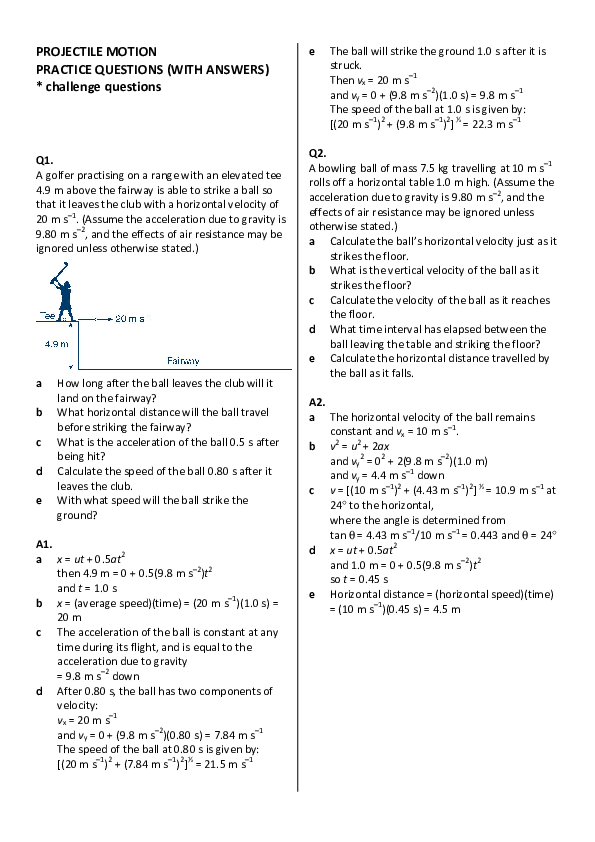## PROJECTILE MOTION PRACTICE QUESTIONS (WITH ANSWERS) * challenge questions

by Hephzibah Akindele

## Free Related PDFs

patrick renison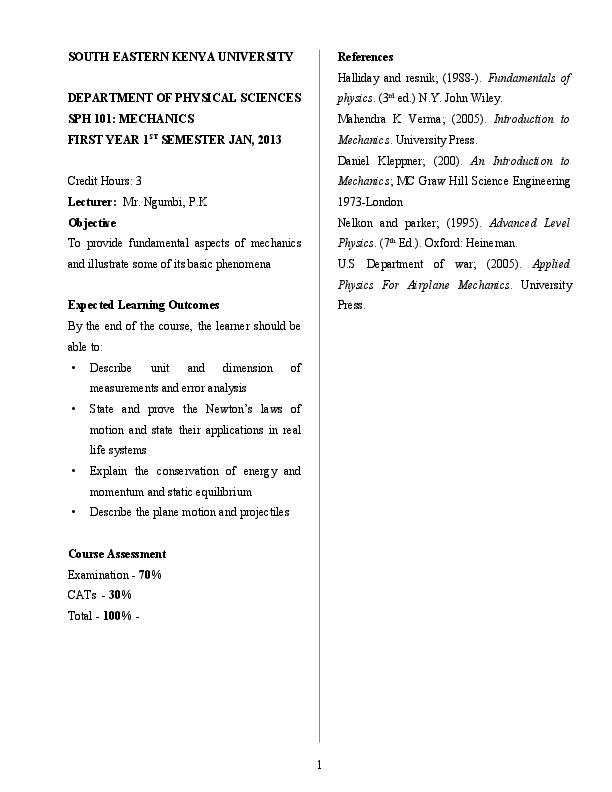Ana Cláudia

resolução do terceiro capitulo do livro Física para cientistas e engenheiros, Tipler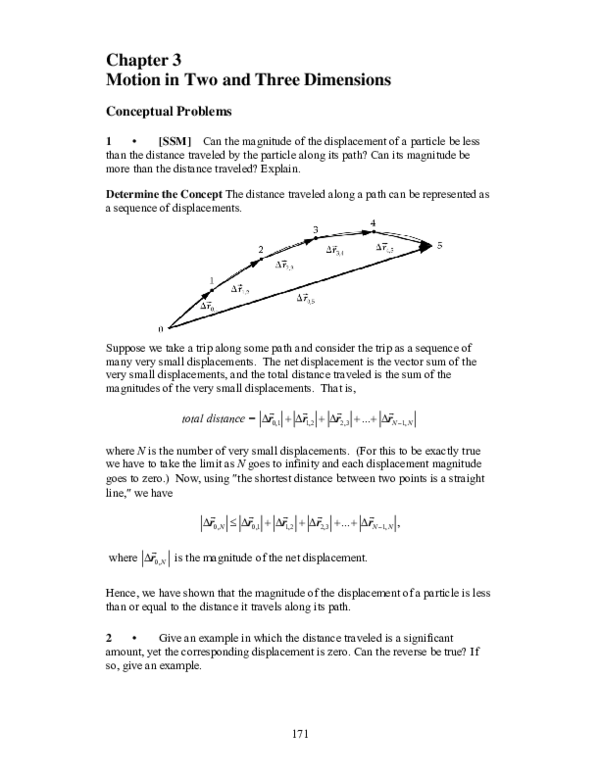Bécquer Amílcar Melgarejo López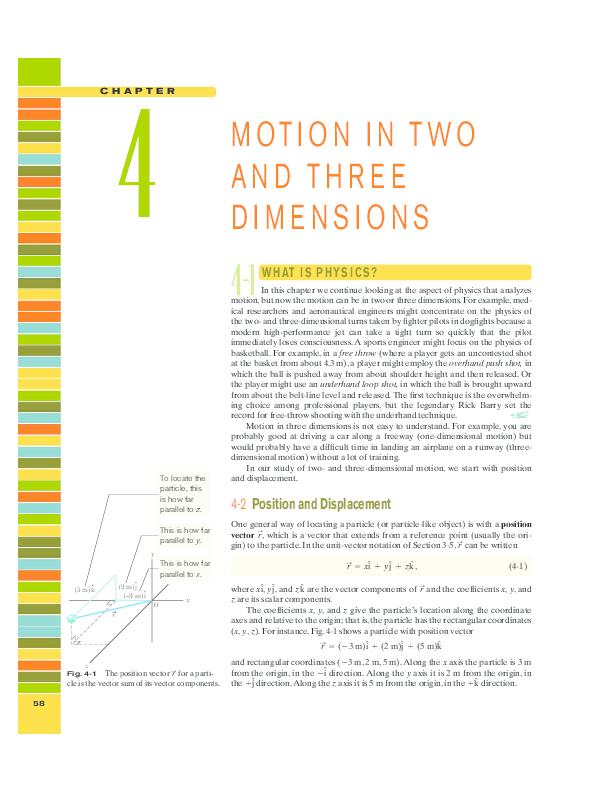Eduardo Endo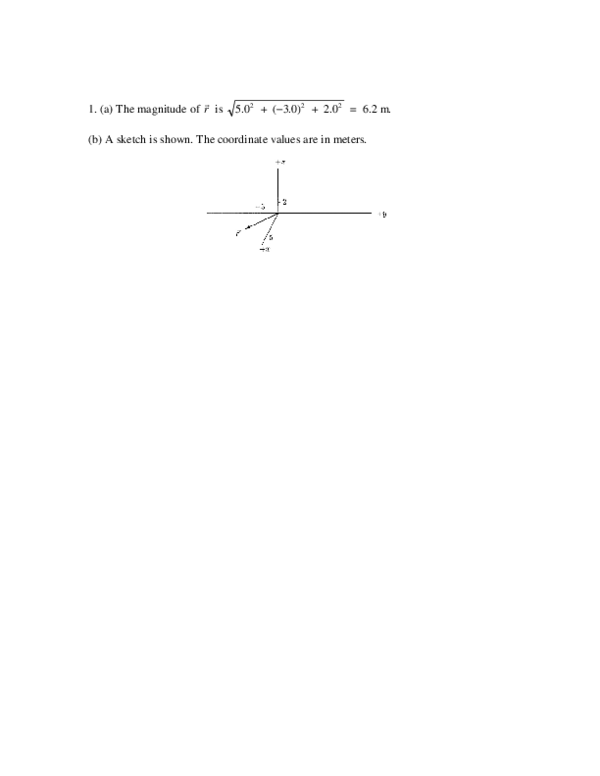Erick Aragon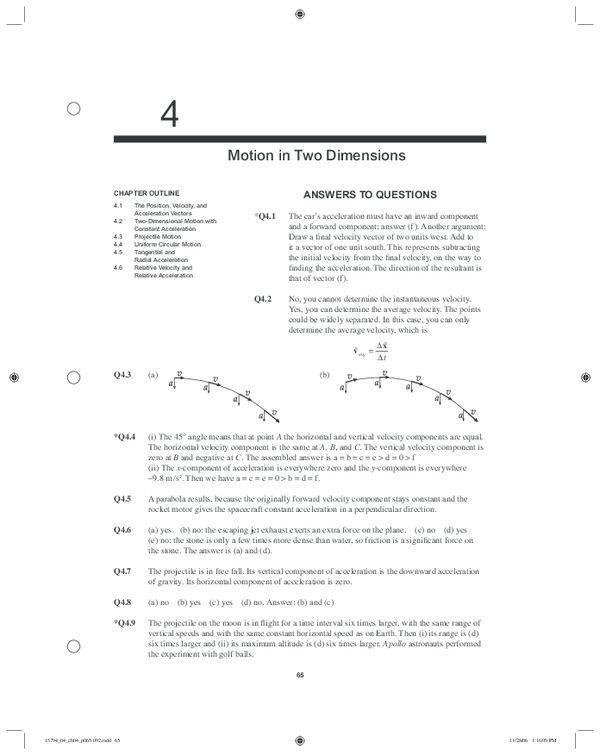ermilson arias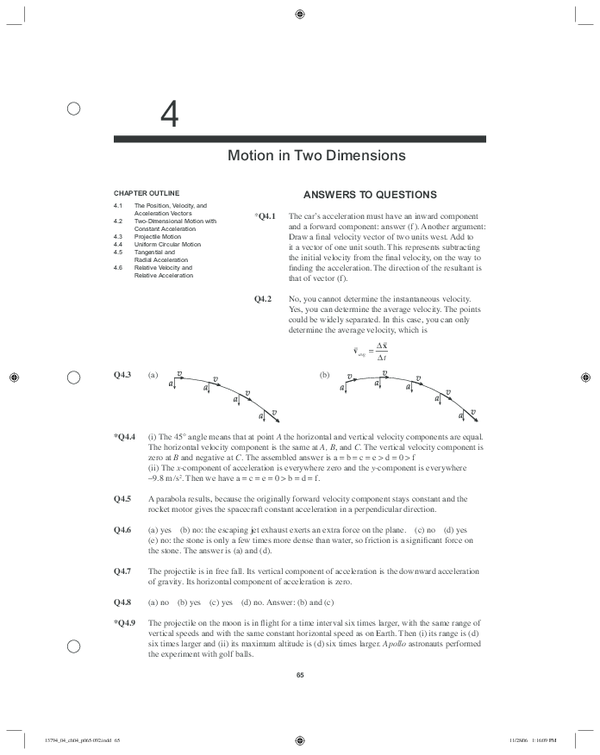Aim To investigate the experimental value of the acceleration due to gravitational force on Earth using projectile motion.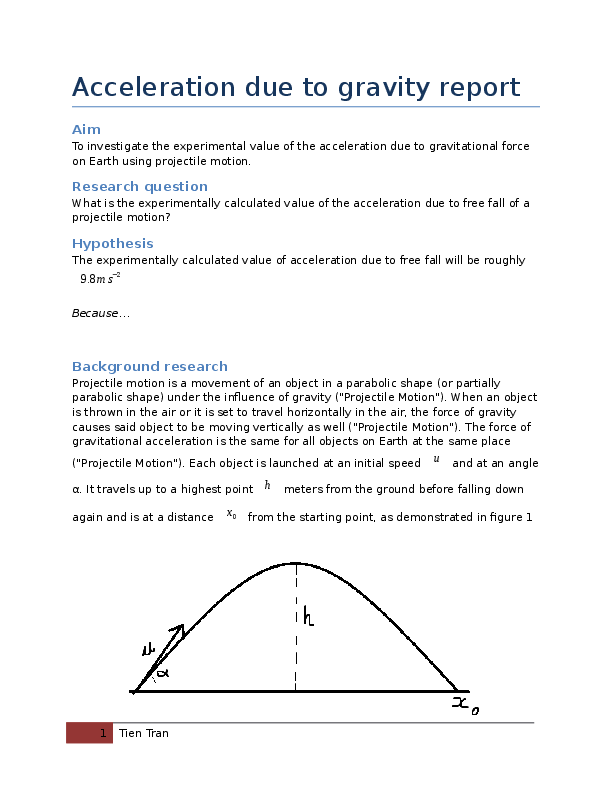Ricardo David Ale Mo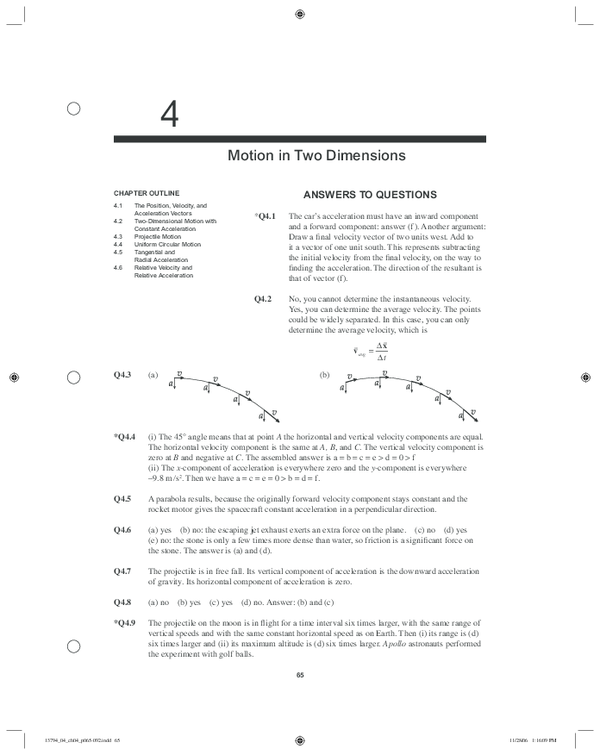•   We're Hiring!
•   Help Center
• Find new research papers in:
• Health Sciences
• Earth Sciences
• Cognitive Science
• Mathematics
• Computer Science

## Physics Problems with Solutions

• Projectile Problems with Solutions and Explanations

Projectile problems are presented along with detailed solutions . These problems may be better understood when projectile equations are first reviewed. An interactive html 5 applet may be used to better understand the projectile equations.

## Problems with Detailed Solutions

An object is launched at a velocity of 20 m/s in a direction making an angle of 25° upward with the horizontal. a) What is the maximum height reached by the object? b) What is the total flight time (between launch and touching the ground) of the object? c) What is the horizontal range (maximum x above ground) of the object? d) What is the magnitude of the velocity of the object just before it hits the ground? Solution to Problem 1

A ball kicked from ground level at an initial velocity of 60 m/s and an angle θ with ground reaches a horizontal distance of 200 meters. a) What is the size of angle θ? b) What is time of flight of the ball? Solution to Problem 5

A ball of 600 grams is kicked at an angle of 35° with the ground with an initial velocity V 0 . a) What is the initial velocity V 0 of the ball if its kinetic energy is 22 Joules when its height is maximum? b) What is the maximum height reached by the ball Solution to Problem 6

A projectile starting from ground hits a target on the ground located at a distance of 1000 meters after 40 seconds. a) What is the size of the angle θ? b) At what initial velocity was the projectile launched? Solution to Problem 7

The trajectory of a projectile launched from ground is given by the equation y = -0.025 x 2 + 0.5 x, where x and y are the coordinate of the projectile on a rectangular system of axes. a) Find the initial velocity and the angle at which the projectile is launched. Solution to Problem 8

Two balls A and B of masses 100 grams and 300 grams respectively are pushed horizontally from a table of height 3 meters. Ball has is pushed so that its initial velocity is 10 m/s and ball B is pushed so that its initial velocity is 15 m/s. a) Find the time it takes each ball to hit the ground. b) What is the difference in the distance between the points of impact of the two balls on the ground? Solution to Problem 9

• Projectile Motion Calculator and Solver
• Solutions and Explanations to Projectile Problems
• Projectile Equations with Explanations
• Interactive Simulation of Projectile .

## Popular Pages

• 5.3 Projectile Motion
• Introduction
• 1.1 Physics: Definitions and Applications
• 1.2 The Scientific Methods
• 1.3 The Language of Physics: Physical Quantities and Units
• Section Summary
• Key Equations
• Concept Items
• Critical Thinking Items
• Multiple Choice
• Extended Response
• 2.1 Relative Motion, Distance, and Displacement
• 2.2 Speed and Velocity
• 2.3 Position vs. Time Graphs
• 2.4 Velocity vs. Time Graphs
• 3.1 Acceleration
• 3.2 Representing Acceleration with Equations and Graphs
• 4.2 Newton's First Law of Motion: Inertia
• 4.3 Newton's Second Law of Motion
• 4.4 Newton's Third Law of Motion
• 5.1 Vector Addition and Subtraction: Graphical Methods
• 5.2 Vector Addition and Subtraction: Analytical Methods
• 5.4 Inclined Planes
• 5.5 Simple Harmonic Motion
• 6.1 Angle of Rotation and Angular Velocity
• 6.2 Uniform Circular Motion
• 6.3 Rotational Motion
• 7.1 Kepler's Laws of Planetary Motion
• 7.2 Newton's Law of Universal Gravitation and Einstein's Theory of General Relativity
• 8.1 Linear Momentum, Force, and Impulse
• 8.2 Conservation of Momentum
• 8.3 Elastic and Inelastic Collisions
• 9.1 Work, Power, and the Work–Energy Theorem
• 9.2 Mechanical Energy and Conservation of Energy
• 9.3 Simple Machines
• 10.1 Postulates of Special Relativity
• 10.2 Consequences of Special Relativity
• 11.1 Temperature and Thermal Energy
• 11.2 Heat, Specific Heat, and Heat Transfer
• 11.3 Phase Change and Latent Heat
• 12.1 Zeroth Law of Thermodynamics: Thermal Equilibrium
• 12.2 First law of Thermodynamics: Thermal Energy and Work
• 12.3 Second Law of Thermodynamics: Entropy
• 12.4 Applications of Thermodynamics: Heat Engines, Heat Pumps, and Refrigerators
• 13.1 Types of Waves
• 13.2 Wave Properties: Speed, Amplitude, Frequency, and Period
• 13.3 Wave Interaction: Superposition and Interference
• 14.1 Speed of Sound, Frequency, and Wavelength
• 14.2 Sound Intensity and Sound Level
• 14.3 Doppler Effect and Sonic Booms
• 14.4 Sound Interference and Resonance
• 15.1 The Electromagnetic Spectrum
• 15.2 The Behavior of Electromagnetic Radiation
• 16.1 Reflection
• 16.2 Refraction
• 16.3 Lenses
• 17.1 Understanding Diffraction and Interference
• 17.2 Applications of Diffraction, Interference, and Coherence
• 18.1 Electrical Charges, Conservation of Charge, and Transfer of Charge
• 18.2 Coulomb's law
• 18.3 Electric Field
• 18.4 Electric Potential
• 18.5 Capacitors and Dielectrics
• 19.1 Ohm's law
• 19.2 Series Circuits
• 19.3 Parallel Circuits
• 19.4 Electric Power
• 20.1 Magnetic Fields, Field Lines, and Force
• 20.2 Motors, Generators, and Transformers
• 20.3 Electromagnetic Induction
• 21.1 Planck and Quantum Nature of Light
• 21.2 Einstein and the Photoelectric Effect
• 21.3 The Dual Nature of Light
• 22.1 The Structure of the Atom
• 22.2 Nuclear Forces and Radioactivity
• 22.3 Half Life and Radiometric Dating
• 22.4 Nuclear Fission and Fusion
• 23.1 The Four Fundamental Forces
• 23.2 Quarks
• 23.3 The Unification of Forces
• A | Reference Tables

## Section Learning Objectives

By the end of this section, you will be able to do the following:

• Describe the properties of projectile motion
• Apply kinematic equations and vectors to solve problems involving projectile motion

## Teacher Support

• (C) analyze and describe accelerated motion in two dimensions using equations.

In addition, the High School Physics Laboratory Manual addresses content in this section in the lab titled: Motion in Two Dimensions, as well as the following standards:

• (C) analyze and describe accelerated motion in two dimensions using equations, including projectile and circular examples.

## Section Key Terms

Properties of projectile motion.

Projectile motion is the motion of an object thrown (projected) into the air when, after the initial force that launches the object, air resistance is negligible and the only other force that object experiences is the force of gravity. The object is called a projectile , and its path is called its trajectory . Air resistance is a frictional force that slows its motion and can significantly alter the trajectory of the motion. Due to the difficulty in calculation, only situations in which the deviation from projectile motion is negligible and air resistance can be ignored are considered in introductory physics. That approximation is often quite accurate.

[BL] [OL] Review addition of vectors graphically and analytically.

[BL] [OL] [AL] Explain the term projectile motion. Ask students to guess what the motion of a projectile might depend on? Is the initial velocity important? Is the angle important? How will these things affect its height and the distance it covers? Introduce the concept of air resistance. Review kinematic equations.

The most important concept in projectile motion is that when air resistance is ignored, horizontal and vertical motions are independent , meaning that they don’t influence one another. Figure 5.27 compares a cannonball in free fall (in blue) to a cannonball launched horizontally in projectile motion (in red). You can see that the cannonball in free fall falls at the same rate as the cannonball in projectile motion. Keep in mind that if the cannon launched the ball with any vertical component to the velocity, the vertical displacements would not line up perfectly.

Since vertical and horizontal motions are independent, we can analyze them separately, along perpendicular axes. To do this, we separate projectile motion into the two components of its motion, one along the horizontal axis and the other along the vertical.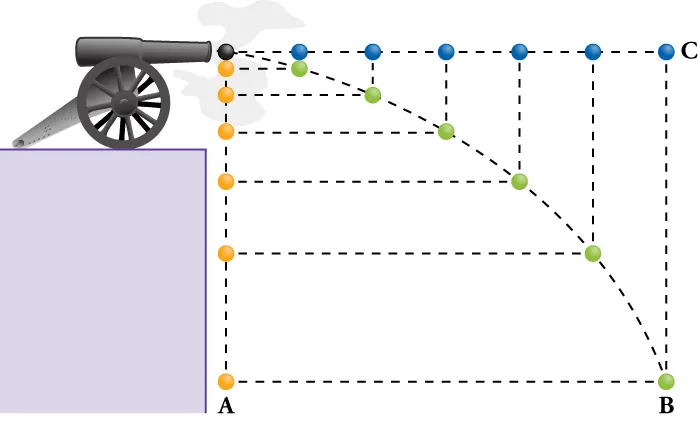We’ll call the horizontal axis the x -axis and the vertical axis the y -axis. For notation, d is the total displacement, and x and y are its components along the horizontal and vertical axes. The magnitudes of these vectors are x and y , as illustrated in Figure 5.28 .As usual, we use velocity, acceleration, and displacement to describe motion. We must also find the components of these variables along the x - and y -axes. The components of acceleration are then very simple a y = – g = –9.80 m/s 2 . Note that this definition defines the upwards direction as positive. Because gravity is vertical, a x = 0. Both accelerations are constant, so we can use the kinematic equations. For review, the kinematic equations from a previous chapter are summarized in Table 5.1 .

Where x is position, x 0 is initial position, v is velocity, v avg is average velocity, t is time and a is acceleration.

## Solve Problems Involving Projectile Motion

The following steps are used to analyze projectile motion:

• Separate the motion into horizontal and vertical components along the x- and y-axes. These axes are perpendicular, so A x = A cos θ A x = A cos θ and A y = A sin θ A y = A sin θ are used. The magnitudes of the displacement s s along x- and y-axes are called x x and y . y . The magnitudes of the components of the velocity v v are v x = v ​ ​ ​ cos θ v x = v ​ ​ ​ cos θ and v y = v ​ ​ ​ sin θ v y = v ​ ​ ​ sin θ , where v v is the magnitude of the velocity and θ θ is its direction. Initial values are denoted with a subscript 0.
• Treat the motion as two independent one-dimensional motions, one horizontal and the other vertical. The kinematic equations for horizontal and vertical motion take the following forms Horizontal Motion ( a x = 0 ) x = x 0 + v x t v x = v 0 x = v x = velocity  is a constant. Horizontal Motion ( a x = 0 ) x = x 0 + v x t v x = v 0 x = v x = velocity  is a constant. Vertical motion (assuming positive is up a y = − g = − 9.80  m/s 2 a y = − g = − 9.80  m/s 2 ) y = y 0 + 1 2 ( v 0 y + v y ) t v y = v 0 y − g t y = y 0 + v 0 y t − 1 2 g t 2 v y 2 = v 0 y 2 − 2 g ( y − y 0 ) y = y 0 + 1 2 ( v 0 y + v y ) t v y = v 0 y − g t y = y 0 + v 0 y t − 1 2 g t 2 v y 2 = v 0 y 2 − 2 g ( y − y 0 )
• Solve for the unknowns in the two separate motions (one horizontal and one vertical). Note that the only common variable between the motions is time t t . The problem solving procedures here are the same as for one-dimensional kinematics.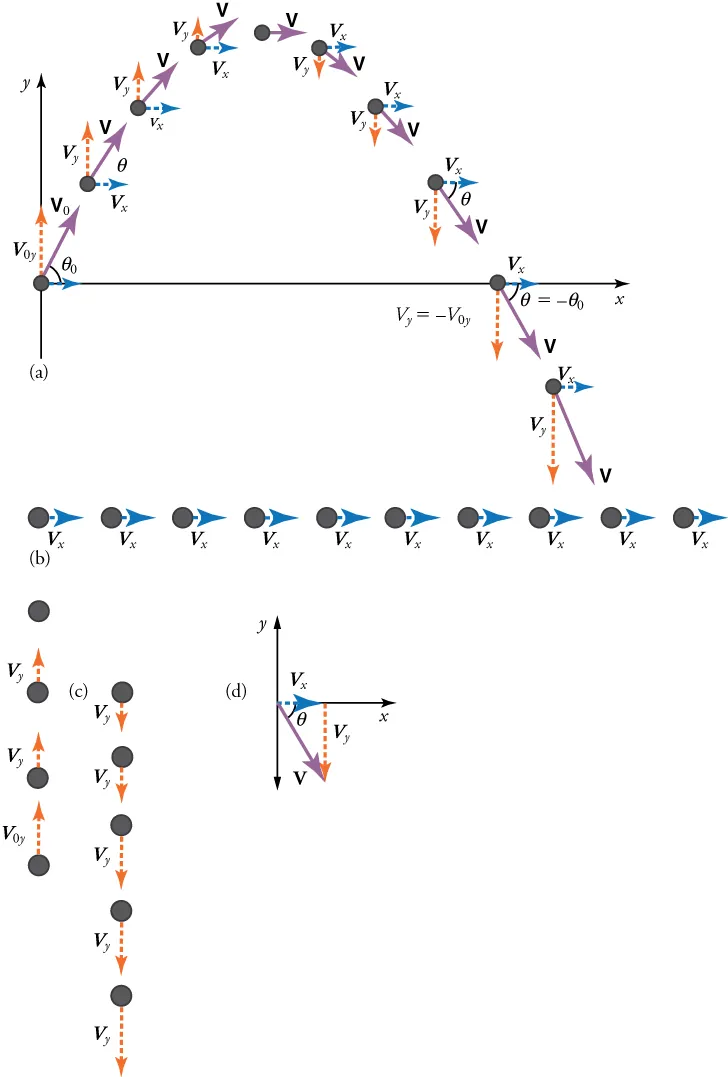## Teacher Demonstration

Demonstrate the path of a projectile by doing a simple demonstration. Toss a dark beanbag in front of a white board so that students can get a good look at the projectile path. Vary the toss angles, so different paths can be displayed. This demonstration could be extended by using digital photography. Draw a reference grid on the whiteboard, then toss the bag at different angles while taking a video. Replay this in slow motion to observe and compare the altitudes and trajectories.

## Tips For Success

For problems of projectile motion, it is important to set up a coordinate system. The first step is to choose an initial position for x x and y y . Usually, it is simplest to set the initial position of the object so that x 0 = 0 x 0 = 0 and y 0 = 0 y 0 = 0 .

## Watch Physics

Projectile at an angle.

This video presents an example of finding the displacement (or range) of a projectile launched at an angle. It also reviews basic trigonometry for finding the sine, cosine and tangent of an angle.

• The time to reach the ground would remain the same since the vertical component is unchanged.
• The time to reach the ground would remain the same since the vertical component of the velocity also gets doubled.
• The time to reach the ground would be halved since the horizontal component of the velocity is doubled.
• The time to reach the ground would be doubled since the horizontal component of the velocity is doubled.

## Worked Example

A fireworks projectile explodes high and away.

During a fireworks display like the one illustrated in Figure 5.30 , a shell is shot into the air with an initial speed of 70.0 m/s at an angle of 75° above the horizontal. The fuse is timed to ignite the shell just as it reaches its highest point above the ground. (a) Calculate the height at which the shell explodes. (b) How much time passed between the launch of the shell and the explosion? (c) What is the horizontal displacement of the shell when it explodes?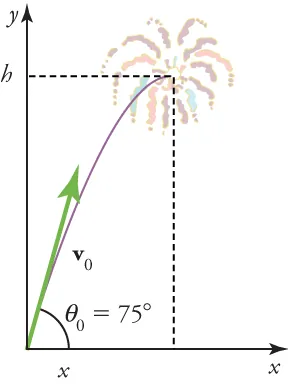The motion can be broken into horizontal and vertical motions in which a x = 0 a x = 0 and   a y = g   a y = g . We can then define x 0 x 0 and y 0 y 0 to be zero and solve for the maximum height .

By height we mean the altitude or vertical position y y above the starting point. The highest point in any trajectory, the maximum height, is reached when   v y = 0   v y = 0 ; this is the moment when the vertical velocity switches from positive (upwards) to negative (downwards). Since we know the initial velocity, initial position, and the value of v y when the firework reaches its maximum height, we use the following equation to find y y

Because y 0 y 0 and v y v y are both zero, the equation simplifies to

Solving for y y gives

Now we must find v 0 y v 0 y , the component of the initial velocity in the y -direction. It is given by v 0 y = v 0 sin θ v 0 y = v 0 sin θ , where v 0 y v 0 y is the initial velocity of 70.0 m/s, and θ = 75 ∘ θ = 75 ∘ is the initial angle. Thus,

Since up is positive, the initial velocity and maximum height are positive, but the acceleration due to gravity is negative. The maximum height depends only on the vertical component of the initial velocity. The numbers in this example are reasonable for large fireworks displays, the shells of which do reach such heights before exploding.

There is more than one way to solve for the time to the highest point. In this case, the easiest method is to use y = y 0 + 1 2 ( v 0 y + v y ) t y = y 0 + 1 2 ( v 0 y + v y ) t . Because y 0 y 0 is zero, this equation reduces to

Note that the final vertical velocity, v y v y , at the highest point is zero. Therefore,

This time is also reasonable for large fireworks. When you are able to see the launch of fireworks, you will notice several seconds pass before the shell explodes. Another way of finding the time is by using y = y 0 + v 0 y t − 1 2 g t 2 y = y 0 + v 0 y t − 1 2 g t 2 , and solving the quadratic equation for t t .

Because air resistance is negligible, a x = 0 a x = 0 and the horizontal velocity is constant. The horizontal displacement is horizontal velocity multiplied by time as given by x = x 0 + v x t x = x 0 + v x t , where x 0 x 0 is equal to zero

where v x v x is the x -component of the velocity, which is given by v x = v 0 cos θ 0 . v x = v 0 cos θ 0 . Now,

The time t t for both motions is the same, and so x x is

The horizontal motion is a constant velocity in the absence of air resistance. The horizontal displacement found here could be useful in keeping the fireworks fragments from falling on spectators. Once the shell explodes, air resistance has a major effect, and many fragments will land directly below, while some of the fragments may now have a velocity in the –x direction due to the forces of the explosion.

[BL] [OL] [AL] Talk about the sample problem. Discuss the variables or unknowns in each part of the problem Ask students which kinematic equations may be best suited to solve the different parts of the problem.

The expression we found for y y while solving part (a) of the previous problem works for any projectile motion problem where air resistance is negligible. Call the maximum height y = h y = h ; then,

This equation defines the maximum height of a projectile . The maximum height depends only on the vertical component of the initial velocity.

## Calculating Projectile Motion: Hot Rock Projectile

Suppose a large rock is ejected from a volcano, as illustrated in Figure 5.31 , with a speed of 25.0   m / s 25.0   m / s and at an angle 3 5 ° 3 5 ° above the horizontal. The rock strikes the side of the volcano at an altitude 20.0 m lower than its starting point. (a) Calculate the time it takes the rock to follow this path.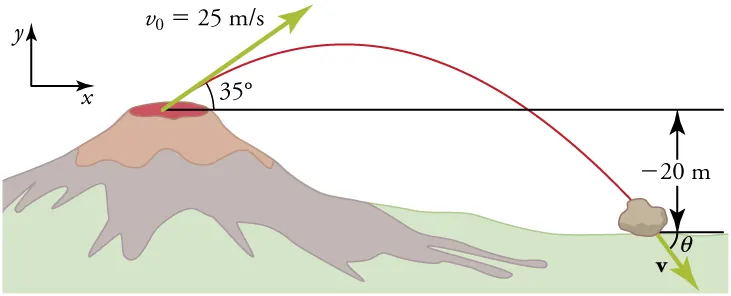Breaking this two-dimensional motion into two independent one-dimensional motions will allow us to solve for the time. The time a projectile is in the air depends only on its vertical motion.

While the rock is in the air, it rises and then falls to a final position 20.0 m lower than its starting altitude. We can find the time for this by using

If we take the initial position y 0 y 0 to be zero, then the final position is y = − 20.0  m . y = − 20.0  m . Now the initial vertical velocity is the vertical component of the initial velocity, found from

Substituting known values yields

Rearranging terms gives a quadratic equation in t t

This expression is a quadratic equation of the form a t 2 + b t + c = 0 a t 2 + b t + c = 0 , where the constants are a = 4.90, b = –14.3, and c = –20.0. Its solutions are given by the quadratic formula

This equation yields two solutions t = 3.96 and t = –1.03. You may verify these solutions as an exercise. The time is t = 3.96 s or –1.03 s. The negative value of time implies an event before the start of motion, so we discard it. Therefore,

The time for projectile motion is completely determined by the vertical motion. So any projectile that has an initial vertical velocity of 14.3 m / s 14.3 m / s and lands 20.0 m below its starting altitude will spend 3.96 s in the air.

## Practice Problems

The fact that vertical and horizontal motions are independent of each other lets us predict the range of a projectile. The range is the horizontal distance R traveled by a projectile on level ground, as illustrated in Figure 5.32 . Throughout history, people have been interested in finding the range of projectiles for practical purposes, such as aiming cannons.How does the initial velocity of a projectile affect its range? Obviously, the greater the initial speed v 0 v 0 , the greater the range, as shown in the figure above. The initial angle θ 0 θ 0 also has a dramatic effect on the range. When air resistance is negligible, the range R R of a projectile on level ground is

where v 0 v 0 is the initial speed and θ 0 θ 0 is the initial angle relative to the horizontal. It is important to note that the range doesn’t apply to problems where the initial and final y position are different, or to cases where the object is launched perfectly horizontally.

## Virtual Physics

Projectile motion.

In this simulation you will learn about projectile motion by blasting objects out of a cannon. You can choose between objects such as a tank shell, a golf ball or even a Buick. Experiment with changing the angle, initial speed, and mass, and adding in air resistance. Make a game out of this simulation by trying to hit the target.

• Projectile motion is the motion of an object projected into the air and moving under the influence of gravity.
• Projectile motion is the motion of an object projected into the air and moving independently of gravity.
• Projectile motion is the motion of an object projected vertically upward into the air and moving under the influence of gravity.
• Projectile motion is the motion of an object projected horizontally into the air and moving independently of gravity.

What is the force experienced by a projectile after the initial force that launched it into the air in the absence of air resistance?

• The nuclear force
• The gravitational force
• The electromagnetic force
• The contact force

Use the Check Your Understanding questions to assess whether students achieve the learning objectives for this section. If students are struggling with a specific objective, the Check Your Understanding will help identify which objective is causing the problem and direct students to the relevant content.

As an Amazon Associate we earn from qualifying purchases.

Want to cite, share, or modify this book? This book uses the Creative Commons Attribution License and you must attribute Texas Education Agency (TEA). The original material is available at: https://www.texasgateway.org/book/tea-physics . Changes were made to the original material, including updates to art, structure, and other content updates.

• Authors: Paul Peter Urone, Roger Hinrichs
• Publisher/website: OpenStax
• Book title: Physics
• Publication date: Mar 26, 2020
• Location: Houston, Texas
• Book URL: https://openstax.org/books/physics/pages/1-introduction
• Section URL: https://openstax.org/books/physics/pages/5-3-projectile-motion

© Jun 24, 2023 Texas Education Agency (TEA). The OpenStax name, OpenStax logo, OpenStax book covers, OpenStax CNX name, and OpenStax CNX logo are not subject to the Creative Commons license and may not be reproduced without the prior and express written consent of Rice University.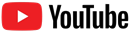• TPC and eLearning
• What's NEW at TPC?
• Practice Review Test
• Teacher-Tools
• Subscription Selection
• Seat Calculator
• Edit Profile Settings
• Student Progress Edit
• Export Student Progress
• Metric Conversions Questions
• Metric System Questions
• Metric Estimation Questions
• Significant Digits Questions
• Proportional Reasoning
• Acceleration
• Distance-Displacement
• Dots and Graphs
• Graph That Motion
• Match That Graph
• Name That Motion
• Motion Diagrams
• Pos'n Time Graphs Numerical
• Pos'n Time Graphs Conceptual
• Up And Down - Questions
• Balanced vs. Unbalanced Forces
• Change of State
• Force and Motion
• Mass and Weight
• Match That Free-Body Diagram
• Net Force (and Acceleration) Ranking Tasks
• Newton's Second Law
• Normal Force Card Sort
• Recognizing Forces
• Air Resistance and Skydiving
• Solve It! with Newton's Second Law
• Which One Doesn't Belong?
• Projectile Mathematics
• Trajectory - Angle Launched Projectiles
• Trajectory - Horizontally Launched Projectiles
• Vector Direction
• Which One Doesn't Belong? Projectile Motion
• Forces in 2-Dimensions
• Explosions - Law Breakers
• Hit and Stick Collisions - Law Breakers
• Case Studies: Impulse and Force
• Impulse-Momentum Change Table
• Keeping Track of Momentum - Hit and Stick
• Keeping Track of Momentum - Hit and Bounce
• What's Up (and Down) with KE and PE?
• Energy Conservation Questions
• Energy Dissipation Questions
• LOL Charts (a.k.a., Energy Bar Charts)
• Match That Bar Chart
• Words and Charts Questions
• Name That Energy
• Stepping Up with PE and KE Questions
• Case Studies - Circular Motion
• Circular Logic
• Forces and Free-Body Diagrams in Circular Motion
• Gravitational Field Strength
• Universal Gravitation
• Angular Position and Displacement
• Linear and Angular Velocity
• Angular Acceleration
• Rotational Inertia
• Balanced vs. Unbalanced Torques
• Getting a Handle on Torque
• Balloon Interactions
• Charge and Charging
• Charge Interactions
• Charging by Induction
• Conductors and Insulators
• Coulombs Law
• Electric Field
• Electric Field Intensity
• Polarization
• Case Studies: Electric Power
• Light Bulb Anatomy
• I = ∆V/R Equations as a Guide to Thinking
• Parallel Circuits - ∆V = I•R Calculations
• Series Circuits - ∆V = I•R Calculations
• Series vs. Parallel Circuits
• Equivalent Resistance
• Period and Frequency of a Pendulum
• Pendulum Motion: Velocity and Force
• Energy of a Pendulum
• Period and Frequency of a Mass on a Spring
• Horizontal Springs: Velocity and Force
• Vertical Springs: Velocity and Force
• Energy of a Mass on a Spring
• Decibel Scale
• Frequency and Period
• Closed-End Air Columns
• Name That Harmonic: Strings
• Rocking the Boat
• Wave Basics
• Matching Pairs: Wave Characteristics
• Wave Interference
• Waves - Case Studies
• Color Filters
• If This, Then That: Color Subtraction
• Light Intensity
• Color Pigments
• Converging Lenses
• Curved Mirror Images
• Law of Reflection
• Refraction and Lenses
• Total Internal Reflection
• Who Can See Who?
• Formulas and Atom Counting
• Atomic Models
• Bond Polarity
• Entropy Questions
• Cell Voltage Questions
• Heat of Formation Questions
• Reduction Potential Questions
• Oxidation States Questions
• Measuring the Quantity of Heat
• Hess's Law
• Oxidation-Reduction Questions
• Galvanic Cells Questions
• Thermal Stoichiometry
• Molecular Polarity
• Quantum Mechanics
• Balancing Chemical Equations
• Bronsted-Lowry Model of Acids and Bases
• Classification of Matter
• Collision Model of Reaction Rates
• Dissociation Reactions
• Complete Electron Configurations
• Enthalpy Change Questions
• Equilibrium Concept
• Equilibrium Constant Expression
• Equilibrium Calculations - Questions
• Equilibrium ICE Table
• Ionic Bonding
• Lewis Electron Dot Structures
• Line Spectra Questions
• Measurement and Numbers
• Metals, Nonmetals, and Metalloids
• Metric Estimations
• Metric System
• Mole Conversions
• Name That Element
• Names to Formulas
• Names to Formulas 2
• Nuclear Decay
• Particles, Words, and Formulas
• Periodic Trends
• Precipitation Reactions and Net Ionic Equations
• Pressure Concepts
• Pressure-Temperature Gas Law
• Pressure-Volume Gas Law
• Chemical Reaction Types
• Significant Digits and Measurement
• States Of Matter Exercise
• Stoichiometry - Math Relationships
• Subatomic Particles
• Spontaneity and Driving Forces
• Gibbs Free Energy
• Volume-Temperature Gas Law
• Acid-Base Properties
• Energy and Chemical Reactions
• Chemical and Physical Properties
• Valence Shell Electron Pair Repulsion Theory
• Writing Balanced Chemical Equations
• Mission CG1
• Mission CG10
• Mission CG2
• Mission CG3
• Mission CG4
• Mission CG5
• Mission CG6
• Mission CG7
• Mission CG8
• Mission CG9
• Mission EC1
• Mission EC10
• Mission EC11
• Mission EC12
• Mission EC2
• Mission EC3
• Mission EC4
• Mission EC5
• Mission EC6
• Mission EC7
• Mission EC8
• Mission EC9
• Mission RL1
• Mission RL2
• Mission RL3
• Mission RL4
• Mission RL5
• Mission RL6
• Mission KG7
• Mission RL8
• Mission KG9
• Mission RL10
• Mission RL11
• Mission RM1
• Mission RM2
• Mission RM3
• Mission RM4
• Mission RM5
• Mission RM6
• Mission RM8
• Mission RM10
• Mission LC1
• Mission RM11
• Mission LC2
• Mission LC3
• Mission LC4
• Mission LC5
• Mission LC6
• Mission LC8
• Mission SM1
• Mission SM2
• Mission SM3
• Mission SM4
• Mission SM5
• Mission SM6
• Mission SM8
• Mission SM10
• Mission KG10
• Mission SM11
• Mission KG2
• Mission KG3
• Mission KG4
• Mission KG5
• Mission KG6
• Mission KG8
• Mission KG11
• Mission F2D1
• Mission F2D2
• Mission F2D3
• Mission F2D4
• Mission F2D5
• Mission F2D6
• Mission KC1
• Mission KC2
• Mission KC3
• Mission KC4
• Mission KC5
• Mission KC6
• Mission KC7
• Mission KC8
• Mission AAA
• Mission SM9
• Mission LC7
• Mission LC9
• Mission NL1
• Mission NL2
• Mission NL3
• Mission NL4
• Mission NL5
• Mission NL6
• Mission NL7
• Mission NL8
• Mission NL9
• Mission NL10
• Mission NL11
• Mission NL12
• Mission MC1
• Mission MC10
• Mission MC2
• Mission MC3
• Mission MC4
• Mission MC5
• Mission MC6
• Mission MC7
• Mission MC8
• Mission MC9
• Mission RM7
• Mission RM9
• Mission RL7
• Mission RL9
• Mission SM7
• Mission SE1
• Mission SE10
• Mission SE11
• Mission SE12
• Mission SE2
• Mission SE3
• Mission SE4
• Mission SE5
• Mission SE6
• Mission SE7
• Mission SE8
• Mission SE9
• Mission VP1
• Mission VP10
• Mission VP2
• Mission VP3
• Mission VP4
• Mission VP5
• Mission VP6
• Mission VP7
• Mission VP8
• Mission VP9
• Mission WM1
• Mission WM2
• Mission WM3
• Mission WM4
• Mission WM5
• Mission WM6
• Mission WM7
• Mission WM8
• Mission WE1
• Mission WE10
• Mission WE2
• Mission WE3
• Mission WE4
• Mission WE5
• Mission WE6
• Mission WE7
• Mission WE8
• Mission WE9
• Vector Walk Interactive
• Name That Motion Interactive
• Kinematic Graphing 1 Concept Checker
• Kinematic Graphing 2 Concept Checker
• Graph That Motion Interactive
• Rocket Sled Concept Checker
• Force Concept Checker
• Free-Body Diagrams Concept Checker
• Free-Body Diagrams The Sequel Concept Checker
• Skydiving Concept Checker
• Elevator Ride Concept Checker
• Vector Walk in Two Dimensions Interactive
• Name That Vector Interactive
• River Boat Simulator Concept Checker
• Projectile Simulator 2 Concept Checker
• Projectile Simulator 3 Concept Checker
• Turd the Target 1 Interactive
• Turd the Target 2 Interactive
• Balance It Interactive
• Go For The Gold Interactive
• Egg Drop Concept Checker
• Fish Catch Concept Checker
• Exploding Carts Concept Checker
• Collision Carts - Inelastic Collisions Concept Checker
• Its All Uphill Concept Checker
• Stopping Distance Concept Checker
• Chart That Motion Interactive
• Roller Coaster Model Concept Checker
• Uniform Circular Motion Concept Checker
• Horizontal Circle Simulation Concept Checker
• Vertical Circle Simulation Concept Checker
• Race Track Concept Checker
• Gravitational Fields Concept Checker
• Orbital Motion Concept Checker
• Balance Beam Concept Checker
• Torque Balancer Concept Checker
• Aluminum Can Polarization Concept Checker
• Charging Concept Checker
• Name That Charge Simulation
• Coulomb's Law Concept Checker
• Electric Field Lines Concept Checker
• Put the Charge in the Goal Concept Checker
• Circuit Builder Concept Checker (Series Circuits)
• Circuit Builder Concept Checker (Parallel Circuits)
• Circuit Builder Concept Checker (∆V-I-R)
• Circuit Builder Concept Checker (Voltage Drop)
• Equivalent Resistance Interactive
• Pendulum Motion Simulation Concept Checker
• Mass on a Spring Simulation Concept Checker
• Particle Wave Simulation Concept Checker
• Boundary Behavior Simulation Concept Checker
• Slinky Wave Simulator Concept Checker
• Simple Wave Simulator Concept Checker
• Wave Addition Simulation Concept Checker
• Standing Wave Maker Simulation Concept Checker
• Painting With CMY Concept Checker
• Stage Lighting Concept Checker
• Filtering Away Concept Checker
• Young's Experiment Interactive
• Plane Mirror Images Interactive
• Who Can See Who Concept Checker
• Optics Bench (Mirrors) Concept Checker
• Name That Image (Mirrors) Interactive
• Refraction Concept Checker
• Total Internal Reflection Concept Checker
• Optics Bench (Lenses) Concept Checker
• Kinematics Preview
• Velocity Time Graphs Preview
• Moving Cart on an Inclined Plane Preview
• Stopping Distance Preview
• Cart, Bricks, and Bands Preview
• Fan Cart Study Preview
• Friction Preview
• Coffee Filter Lab Preview
• Friction, Speed, and Stopping Distance Preview
• Up and Down Preview
• Projectile Range Preview
• Ballistics Preview
• Juggling Preview
• Marshmallow Launcher Preview
• Air Bag Safety Preview
• Colliding Carts Preview
• Collisions Preview
• Engineering Safer Helmets Preview
• Energy on an Incline Preview
• Modeling Roller Coasters Preview
• Hot Wheels Stopping Distance Preview
• Ball Bat Collision Preview
• Energy in Fields Preview
• Weightlessness Training Preview
• Roller Coaster Loops Preview
• Universal Gravitation Preview
• Keplers Laws Preview
• Kepler's Third Law Preview
• Charge Interactions Preview
• Sticky Tape Experiments Preview
• Wire Gauge Preview
• Voltage, Current, and Resistance Preview
• Light Bulb Resistance Preview
• Series and Parallel Circuits Preview
• Thermal Equilibrium Preview
• Linear Expansion Preview
• Heating Curves Preview
• Electricity and Magnetism - Part 1 Preview
• Electricity and Magnetism - Part 2 Preview
• Vibrating Mass on a Spring Preview
• Period of a Pendulum Preview
• Wave Speed Preview
• Standing Waves in a Rope Preview
• Sound as a Pressure Wave Preview
• DeciBel Scale Preview
• DeciBels, Phons, and Sones Preview
• Sound of Music Preview
• Shedding Light on Light Bulbs Preview
• Models of Light Preview
• Electromagnetic Spectrum Preview
• EM Wave Communication Preview
• Digitized Data Preview
• Light Intensity Preview
• Concave Mirrors Preview
• Object Image Relations Preview
• Snells Law Preview
• Reflection vs. Transmission Preview
• Magnification Lab Preview
• Reactivity Preview
• Ions and the Periodic Table Preview
• Periodic Trends Preview
• Gaining Teacher Access
• Subscription
• Subscription Locator
• 1-D Kinematics
• Newton's Laws
• Vectors - Motion and Forces in Two Dimensions
• Momentum and Its Conservation
• Work and Energy
• Circular Motion and Satellite Motion
• Thermal Physics
• Static Electricity
• Electric Circuits
• Vibrations and Waves
• Sound Waves and Music
• Light and Color
• Reflection and Mirrors
• Usage Policy
• Newtons Laws
• Vectors and Projectiles
• Forces in 2D
• Momentum and Collisions
• Circular and Satellite Motion
• Balance and Rotation
• Waves and Sound
• 1-Dimensional Kinematics
• Circular, Satellite, and Rotational Motion
• Einstein's Theory of Special Relativity
• Waves, Sound and Light
• QuickTime Movies
• Forces in Two Dimensions
• Work, Energy, and Power
• Circular Motion and Gravitation
• Sound Waves
• Pricing For Schools
• Directions for Version 2
• Measurement and Units
• Relationships and Graphs
• Rotation and Balance
• Vibrational Motion
• Reflection and Refraction
• Teacher Accounts
• Kinematic Concepts
• Kinematic Graphing
• Wave Motion
• Sound and Music
• 1D Kinematics
• Vectors and Forces in 2D
• Simple Harmonic Motion
• Rotational Kinematics
• Rotation and Torque
• Rotational Dynamics
• Electric Fields, Potential, and Capacitance
• Transient RC Circuits
• Electromagnetism
• Light Waves
• Units and Measurement
• Stoichiometry
• Molarity and Solutions
• Thermal Chemistry
• Acids and Bases
• Kinetics and Equilibrium
• Solution Equilibria
• Oxidation-Reduction
• Nuclear Chemistry
• NGSS Alignments
• 1D-Kinematics
• Projectiles
• Circular Motion
• Magnetism and Electromagnetism
• Graphing Practice
• ACT Preparation
• For Teachers
• Other Resources
• Newton's Laws of Motion
• Work and Energy Packet
• Static Electricity Review
• Solutions Guide
• Motion in One Dimension
• Work, Energy and Power
• NGSS Search
• Force and Motion DCIs - High School
• Energy DCIs - High School
• Wave Applications DCIs - High School
• Force and Motion PEs - High School
• Energy PEs - High School
• Wave Applications PEs - High School
• Crosscutting Concepts
• The Practices
• Physics Topics
• NGSS Corner: Activity List
• NGSS Corner: Infographics
• Position-Velocity-Acceleration
• Position-Time Graphs
• Velocity-Time Graphs
• Newton's First Law
• Newton's Second Law
• Newton's Third Law
• Terminal Velocity
• Projectile Motion
• Forces in 2 Dimensions
• Impulse and Momentum Change
• Momentum Conservation
• Work-Energy Fundamentals
• Work-Energy Relationship
• Roller Coaster Physics
• Satellite Motion
• Electric Fields
• Circuit Concepts
• Series Circuits
• Parallel Circuits
• Describing-Waves
• Wave Behavior Toolkit
• Standing Wave Patterns
• Resonating Air Columns
• Wave Model of Light
• Plane Mirrors
• Curved Mirrors
• Teacher Guide
• Using Lab Notebooks
• Current Electricity
• Light Waves and Color
• Reflection and Ray Model of Light
• Refraction and Ray Model of Light
• Teacher Resources
• Subscriptions• Newton's Laws
• Einstein's Theory of Special Relativity
• School Pricing
• Newton's Laws of Motion
• Newton's First Law
• Newton's Third Law

## Mechanics: Vectors and Projectiles

Calculator pad, version 2, vectors and projectiles: problem set.

Coach Sweeney walks 26 yards to the north along the sideline, pauses, and walks 12 yards back to the south.

a. Determine the distance which Coach moved. b. Determine Coach's resultant displacement.

• Audio Guided Solution

a. Rosa Boat is paddling upstream at 1.25 m/s relative to the water in a river which is flowing at 0.50 m/s relative to the banks of the river. What is the resultant velocity of Rosa's boat (relative to the banks)? b. Rosa Boat is paddling downstream at 1.25 m/s relative to the water in a river which is flowing at 0.50 m/s relative to the banks of the river. What is the resultant velocity of Rosa's boat (relative to the banks)?

The takeoff speed of a military aircraft from an aircraft carrier is approximately 170 mi/hr relative to the air. They acquire this speed through a combination of a catapult system present on the aircraft carrier and the aircraft's jet propulsion system. A common strategy is to head the carrier and the plane into the wind. If a plane is taking off from an aircraft carrier which is moving at 40 mi/hr into a 20 mi/hr headwind, then what speed relative to the deck of the aircraft carrier must it obtain to takeoff?

Claire de Iles is shopping. She walks 16 m to the end of an aisle. She then makes a right hand turn and walks 21 m down the end aisle. Determine the magnitude of Claire's resultant displacement.

Jim Nazium is walking from lunch to his PE class. He exits the lunchroom and walks 43 m west. He then turns and walks 72 m north down the hallway leading to the locker room. Determine the magnitude and direction of Jim's resultant displacement.

On her trip from home to school, Karla drives along three streets after exiting the driveway. She drives 1.85 miles south, 2.43 miles east and 0.35 miles north. Determine the magnitude of Karla's resultant displacement.

Sheila is captain of the Varsity cross country team. During the after-school practice on Tuesday, she led the team on the following run from school to a nearby park where they met the coach for a meeting: 0.68 miles, north; 1.09 miles east; 1.56 miles north; 0.32 miles, west. Determine the magnitude and direction of the team's resultant displacement.

During the Vector Addition lab, Mac and Tosh start at the classroom door and walk 40.0 m, north, 32.5 m east, 15.5 m south, 68.5 m west, and 2.5 m, north. Determine the magnitude and direction of the resultant displacement of Mac and Tosh.

Avery, the quarterback of South's Varsity football team, made the most amazing pass in the Homecoming game against cross town rival North. He threw the pass from the exact center of the field to the corner of the end zone, where Jamaal caught it for the game winning score. If the football field is 160 feet wide (sideline to sideline) and it is 60 yards from midfield to the back of the end zone, then how far did the ball travel from Avery's hands to Jamaal's hands.

## Problem 10:

Consider the map of the United States below. Given the scale that 1 cm = 340 km, a protractor and a ruler can be used to determine the magnitude and direction for the following trips. All directions are expressed using the counter-clockwise from east convention. For each trip, use the sine, cosine and tangent functions to determine the horizontal and vertical components of the displacement. Be sure to indicate E, W, N, or S as the direction for each component.

## Problem 11:

The pilot of a plane flying due north is notified by the flight controller that there is a second plane flying south at about the same altitude and located in the same general area. The pilot is told that the southward bound plane is currently located at a position which is 13.5 km, 102° from her own plane.

a. How many kilometers to the north is the second plane? b. How many kilometers to the west is the second plane? c. If the two planes both have an airspeed of 290. km/hr, then how much time will elapse before the planes are side by side ?

## Problem 12:

A spelunker (person who explores caves) determines that the cave entrance is located 349 m, 253° from her current position. How far south and how far west from her current position is the cave entrance?## Problem 13:

Avery, South's quarterback, throws a pass 36.5 yards at 21° W of S before it is caught by Mitchell with a diving catch. Assuming that the field runs north and south, and that Avery threw the pass from 7.2 yards behind the line of scrimmage, how many yards were gained on the play?

## Problem 14:

Mia Ander exits the front door of her home and walks along the path shown in the diagram at the right (not to scale). The walk consists of four legs with the following magnitudes:

A = 88 m B = 272 m C = 136 m D = 183 m

Determine the magnitude and direction of Mia's resultant displacement.

## Problem 15:

Dora is exploring a cave. She starts at the entrance and makes the following straight line movements:

68 m, south 112 m, 25° north of west (155° CCW) 34 m, south 182 m, 17° south of east (343° CCW)

Determine Dora's position relative to the entrance of the cave. That is, how far and in what direction is Dora from the cave entrance?

## Problem 16:

Taylor and Drew finish their last class on the day before Spring break and decide to take a spontaneous road trip. Their trip involves the following movements:

42 miles, 67° north of west (113° CCW) 61 miles, west 23 miles, 17° west of south (253° CCW)

Taylor's car breaks down after the last leg of the trip. How far and in what direction are Taylor and Drew from campus?

## Problem 17:

A weather report shows that a tornado was sighted 12 km south and 23 km west of your town. The storm is reported to be moving directly towards your town at a speed of 82 km/hr.

a. What distance from your town was the tornado sighted? b. Approximately how much time (in minutes and hours) will elapse before the violent storm arrives at your town?

## Problem 18:

An airplane begins its journey into Canada from a destination located 285 mi south of the border. The plane flies along a straight-line path at 189 mi/h in a direction of 20.5 degrees west of north. Determine the number of minutes before the plane crosses the border. Assume that the border is aligned directly east and west in the region where the flight takes place.

## Problem 19:

Glenda and Harold are attempting to cross a river in a kayak. The river flows due east at 1.9 m/s. Glenda and Harold head the kayak due north and row at 2.4 m/s (relative to the water). The river is 38 m wide at this location.

a. Determine the resultant velocity of the boat - both magnitude and direction. b. Determine the time for Glenda and Harold to cross the river. c. How far downstream will the boat be when Glenda and Harold reach the opposite shore?

## Problem 20:

Ty Ridlegs boards a paddle boat and heads the boat westward directly across a river. The river flows south at 48 cm/s. Ty paddles the boat with a speed of 98 cm/s.

a. Determine the resultant velocity of the boat - both magnitude and direction. b. If the river is 22 m wide at this location, then how much time does it take Ty to cross the river? Assume that Ty keeps his paddle boat headed west. c. How far downstream will Ty be when he reaches the other side of the river?

## Problem 21:

Dylan and Sophia are walking along Bluebird Lake on a perfectly calm day. Dylan, determined to impress Sophia by his ability to skip rocks, picks up the flattest rock he can find and gives it a sidearm launch from the edge of the water. The rock acquires a completely horizontal velocity of 26 m/s from a height of 0.45 m above the water surface.

a. How much time does it take the rock to fall to the water surface?

## Problem 22:

In an effort to create a cannonball-style splash, eight-year old Matthew runs off the edge of the board of the high dive at 4.6 m/s and falls 2.3 m to the water below.

a. Determine the time for Matthew to fall the 2.3 m to the water. b. What horizontal distance from the edge of the board will Matthew plunge into the water? c. With what speed does Matthew enter the water?

## Problem 23:

Ima Peode wishes to throw a 2.8-kg pumpkin horizontally off the top of the school roof in order to hit Mr. H's car. The car is parked a distance of 13.4 m away from the base of the building below the point where Ima is standing. The building's roof is 10.4 m high. Assuming no air resistance, with what horizontal speed must Ima toss the pumpkin in order to hit Mr. H's car.

## Problem 24:

The La Quebrada Cliff Divers provide daily entertainment for the crowds at Acapulco, Mexico. As a group of professional high divers, they dive off the cliff of La Quebrada and fall 45.1 m (148 feet) to the water below. More than an act of bravery, the cliff divers must time their dive so that they hit the water when the crest of an incoming wave has arrived. Determine the speed with which Pedro must run off the cliff in order to land in the water a horizontal distance of 17.8 m from the edge of the cliff.

## Problem 25:

An emergency relief plane is dropping a care package from a plane to a group of medical personnel working for a relief agency in an African village. The package is designed to land in a small lake, inflate an attached raft upon impact, and finally resurface with the raft side down. The plane will be moving horizontally with a ground speed of 59.1 m/s. The package will be dropped a horizontal distance of 521 m from the intended target location. At what altitude above the pond must the plane be flying in order to successfully accomplish this feat?

## Problem 26:

The Choo Choo Restaurant in DesPlaines, IL is a 50’s style diner which is notorious for the delivery of food from the kitchen to the dining room by an O-scale model train. Dinner baskets filled with hot dogs, hamburgers, French fries and the like are mounted to the tops of flatbed train cars and transported to table tops. On Matthew’s fifth birthday, a French fry rolled off the top of the pile on a tight turn moving at a speed of 1.25 m/s and fell to the floor.

a. Determine the time for the French fry to fall 113 cm from the top of the pile to the floor. b. Determine the horizontal displacement of the fry from the edge of the track. c. Determine the speed of the French fry upon striking the floor.

## Problem 27:

Aaron Agin and Bud Derfenger are lab partners who last year earned a reputation for breaking beakers, spilling acid, mixing the wrong chemicals, breaking thermometers and accidentally lighting Sophia’s hair on fire with a Bunsen burner. And now to the delight of the physics class, Mr. H has made the mistake of allowing them to partner again. In a recent lab which utilized expensive tracks and carts, Aaron and Bud lived up to their reputation. Despite strong warnings from Mr. H, they allowed a cart to roll off the track and then off the table with a speed of 208 cm/s. The crash of the cart to the floor a horizontal distance of 96.3 cm from the table’s edge turned the entire classroom silent. Use this information to determine the height of the lab tables in Mr. H’s lab.

## Problem 28:

Sharon Steady and Al Wayskachon won South’s recent egg toss contest held during Homecoming week. In their winning toss, Sharon gave the egg an underhand toss, releasing it with a velocity of 8.06 m/s at an angle of 30° to the horizontal. To the pleasure of the crowd, Al caught the egg at the same height as the toss without even a fracture to its shell.

a. Calculate the horizontal and vertical components of the initial velocity. b. Calculate the time for the egg to reach the midpoint of the trajectory. c. Calculate the total time the egg is in the air. d. Calculate the horizontal distance which the egg traveled from Sharon to Al. e. Calculate the height of the egg (relative to the releast point) when it was at the peak of its trajectory.

## Problem 29:

Li Ping Phar, the famous Chinese ski jumper, leaves the ramp with an initial velocity of 34.9 m/s at an angle of 35°.

a. Determine the total time of flight. b. Determine the horizontal displacement. c. Determine the peak height (relative to the starting height). Assume that Li lands at the same height as the top of the ramp and that Li is a projectile.

## Problem 30:

A tennis player stretches out to reach a ball that is just barely above the ground and successfully 'lobs' it over her opponent's head. The ball is hit with a speed of 18.7 m/s at an angle of 65.1 degrees.

a. Determine the time that the ball is in the air. b. Determine the maximum height which the ball reaches. c. Determine the distance the ball travels horizontally before landing.

## Problem 31:

On New Year’s eve of 2007, Robbie Maddison set the world record for the longest motorcycle jump, traveling 98.3 m through the air from ramp to ramp. (The record has since been broken several times by Maddision himself.) Assuming a launch angle of 45°, insignificant air resistance and a landing location at the same height as the launch height, determine the speed with which Maddison left the ramp.

## Problem 32:

Mr. Udadi takes his three children to the park for some summertime recreation. Olive Udadi is enjoying swinging and jumping. On one jump, Olive leaves the swing at a 30° angle to the horizontal with a speed of 2.2 m/s. She lands on the ground a horizontal distance of 1.09 m from the launch location.

a. Determine the horizontal and the vertical components of the initial velocity. b. Determine the time which Olive is in the air. c. Determine the vertical height (relative to the landing location) from which Olive jumps from the swing.

## Problem 33:

In an apparent effort to earn an appearance on the Destroyed in Seconds show, Caleb attempts a bicycle maneuver in which he jumps between two ramps whose elevated edges are located a distance of 1.8 meters apart. The ramps are angled at 35° and located at the same height. Determine the speed (in m/s and mi/hr) that Caleb must acquire to accomplish this stunt. (Given: 1.00 m/s = 2.24 mi/hr)

## Problem 34:

Albert is South’s star punter for the varsity football team. His best hang time this past season was for a punt which he kicked at 74° above the horizontal. The punt had a 6.2 second hang time.

a. Determine the speed at which the ball was punted. b. Determine the horizontal distance which the ball traveled.

## View Audio Guided Solution for Problem:

1 | 2 | 3 | 4 | 5 | 6 | 7 | 8 | 9 | 10 | 11 | 12 | 13 | 14 | 15 | 16 | 17 | 18 | 19 | 20 | 21 | 22 | 23 | 24 | 25 | 26 | 27 | 28 | 29 | 30 | 31 | 32 | 33 | 34

Gurumuda Networks

## Projectile motion – problems and solutions

27 Projectile motion – problems and solutions

1. A bullet fired a t an angle θ = 60 o with a velocity of 20 m/s. Acceleration due to gravity is 10 m/s 2 . What is the time interval to reach the maximum height?

The initial velocity of bullet (v o ) = 20 m/s

Angle (θ) = 60 o C

Acceleration due to gravity (g) = 10 m s –2

Wanted : The time interval to reach the maximum height

The initial velocity at the horizontal direction (x axis) :

v ox = v o cos 60 o = (20)(0.5) = 10 m/s

The initial velocity at the vertical direction (y axis) :

v oy = vo sin 60 o = (20)(0.5√3) = 10√3 m/s

The time interval to reach the maximum height, calculated using this equation :

v ty = v oy + g t

v ty = the final velocity in the vertical direction = the final velocity at the highest point = 0 m/s

v oy = the initial velocity at the horizontal direction = 10√3 m/s

g = acceleration due to gravity = 10 m/s 2

t = time interval

The time interval :

0 = 10√3 – 10 t

10√3 = 10 t

t = 10√3 / 10

t = √3 seconds

2. An object projected at an angle. The height of the object is the same when the time interval = 1 second and 3 seconds. What is the time interval the object in air.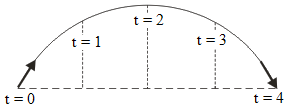The object in the air for 4 seconds.

3. An aircraft is moving horizontally with a speed of 50 m/s. At the height of 2 km, an object is dropped from the aircraft. Acceleration due to gravity = 10 m/ s2, what is the time interval before the object hits the ground.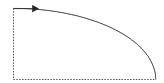Acceleration due to gravity (g) = 10 m/s 2

Wanted : The time interval (t)

h = 1/2 g t 2

2000 = 1/2 (10) t 2

2000 = 5 t 2

t 2 = 2000/5 = 400

t = √400 = 20 seconds

4. A kicked football leaves the ground at an angle θ = 45 o with the horizontal has an initial speed of 25 m/s. Determine the distance of X. Acceleration due to gravity is 10 m/s 2 .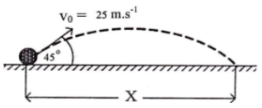Initial speed (v o ) = 25 m/s

Angle (θ) = 45 o

The horizontal component of the initial velocity :

v ox = v o cos θ = (25 m/s)(cos 45 o ) = (25 m/s)(0.5√2) = 12.5√2 m/s

The vertical component of the initial velocity :

v oy = v o sin θ = (25 m/s)(sin 45 o ) = (25 m/s)(0.5√2) = 12.5√2 m/s

Projectile motion could be understood by analyzing the horizontal and vertical component of the motion separately. The x motion occurs at constant velocity and the y motion occurs at constant acceleration of gravity.

Time in the air (t) :

The time in air calculated with the equation of the upward vertical motion.

Choose upward direction as positive and downward direction as negative.

The initial velocity (v o ) = 12.5√2 m/s (upward direction, positive)

Acceleration due to gravity (g) = -10 m/s 2 (downward direction, negative)

Height (h) = 0

Wanted : Time interval (t)

h = v o t + 1/2 g t 2

0 = (12.5√2) t + 1/2 (-10) t 2

0 = 12.5√2 t – 5 t 2

12.5√2 t = 5 t 2

12.5√2 = 5 t

t = 12.5√2 / 5

t = 2.5√2 seconds

The horizontal distance (X) :

Calculated using the equation of the uniform linear motion with constant velocity.

Velocity (v) = 12.5√2 m/s

Time interval (t) = 2.5√2 seconds

Wanted : Distance

d = v t = (12.5√2)(2.5√2) = (12.5)(2.5)(2) = 62.5 meters

5. An object projected upward at an angle θ = 30 o with the horizontal has an initial speed of 20 m/s. Acceleration due to gravity is 10 m/s 2 . Determine the maximum height.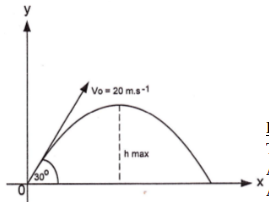Angle (θ) = 30 o

Wanted : The maximum height

First, find the vertical component of the initial velocity (v oy ) :

v oy = v o sin 30 o = (20)(sin 30 o ) = (20)(0.5) = 10 m/s

Calculate the maximum height. Choose upward direction as positive and downward direction as negative.

Acceleration due to gravity (g) = -10 m/s 2 ( downward direction, negative )

The vertical component of the initial velocity (v oy ) = 10 m/s ( upward direction, positive )

Velocity at the maximum height (v ty ) = 0

Wanted : The maximum height (h)

v t 2 = v o 2 + 2 g h

0 2 = 10 2 + 2 (-10) h

0 = 100 – 20 h

h = 5 meters

The maximum height is 5 meters.

6. An object is thrown at a certain elevation angle. The height of the object same after 1 second and 3 seconds. Determine time in air.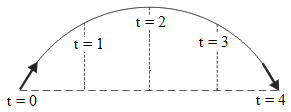Time in air = 4 seconds.

7. An aircraft is moving horizontally with the speed of 50 m/s. When the aircraft at the height of 2 km, an object free fall from the aircraft. Determine the type of the motion.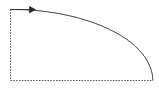B. Floating motion

C. Horizontal motion

D. Projectile motion

The object is dropped from the moving plane because it has the same speed as the plane’s speed, that is 50 m/s. Movement of objects is not like free fall motion but parabolic motion. The case is the same as you are dropping objects from inside a moving car.

8. A ball is thrown horizontally at 15 m/s from a cliff 60 meters high. How long does it take to hit the ground? Solution: Using $$h = \frac{1}{2} g t^2$$, we find the time is $$t = \sqrt{\frac{2h}{g}} \approx 3.5\ \text{s}$$.

9. A projectile is fired at an angle of 30° above the horizontal with an initial speed of 20 m/s. What is the maximum height reached? Solution: Using $$h = \frac{v^2 \sin^2 \theta}{2g}$$, the maximum height is $$h \approx 10.2\ \text{m}$$.

10. A stone is thrown horizontally at 10 m/s from a tower 80 meters tall. Find the horizontal distance it travels before hitting the ground. Solution: Using the time found in a similar way to Problem 1, the horizontal distance is $$d = vt \approx 40\ \text{m}$$.

11. A cannonball is fired at 40 m/s at an angle of 45°. Find the time of flight. Solution: Using $$t = \frac{2v \sin \theta}{g}$$, the time of flight is $$t \approx 5.8\ \text{s}$$.

12.  A baseball is thrown at an angle of 60° with a velocity of 12 m/s. Find the horizontal range. Solution: Using $$R = \frac{v^2 \sin 2\theta}{g}$$, the range is $$R \approx 14.0\ \text{m}$$.

13. A projectile is launched with an initial velocity of 50 m/s at 37° above the horizontal. What is the vertical velocity component? Solution: The vertical component is $$v_y = v \sin \theta \approx 30\ \text{m/s}$$.

14. A projectile is launched horizontally at 20 m/s from a height of 100 meters. What is the vertical velocity just before it hits the ground? Solution: Using $$v_y = \sqrt{2gh}$$, the vertical velocity is $$v_y \approx 44.7\ \text{m/s}$$.

15. A rock is thrown at an angle of 25° above the horizontal with an initial speed of 15 m/s. What are the horizontal and vertical components of the velocity? Solution: The horizontal component is $$v_x = v \cos \theta \approx 13.4\ \text{m/s}$$, and the vertical component is $$v_y \approx 6.4\ \text{m/s}$$.

16. A soccer ball is kicked with an initial speed of 30 m/s at an angle of 40° above the horizontal. What is its horizontal velocity component? Solution: The horizontal component is $$v_x = v \cos \theta \approx 22.9\ \text{m/s}$$.

17. A golf ball is hit with an initial speed of 70 m/s at an angle of 20°. What is the time of flight? Solution: Using the time of flight equation, the time is $$t \approx 4.9\ \text{s}$$.

18. A projectile is fired from the ground with a speed of 25 m/s at 53° above the horizontal. What is its initial vertical velocity component? Solution: The vertical component is $$v_y = v \sin \theta \approx 20\ \text{m/s}$$.

19. A baseball is thrown with an initial speed of 20 m/s at an angle of 50°. What is the maximum height? Solution: Using the equation for maximum height, the height is $$h \approx 15.3\ \text{m}$$.

20. A bullet is fired horizontally with a velocity of 200 m/s from a height of 10 meters. How long does it take to hit the ground? Solution: Using the time equation, the time is $$t \approx 1.4\ \text{s}$$.

21. A cannonball is fired at 45 m/s at an angle of 30°. Find the range. Solution: Using the range equation, the range is $$R \approx 88.2\ \text{m}$$.

22. A basketball is thrown at an angle of 75° with a velocity of 10 m/s. Find the horizontal range. Solution: Using the range equation, the range is $$R \approx 5.3\ \text{m}$$.

23. A projectile is launched with an initial velocity of 30 m/s at 22° above the horizontal. What is the vertical velocity just before it hits the ground? Solution: Using the vertical velocity equation, the vertical velocity is $$v_y \approx 11.4\ \text{m/s}$$.

24. A rock is thrown horizontally at 8 m/s from a tower 40 meters tall. What is the horizontal distance it travels? Solution: Using the horizontal distance equation, the distance is $$d \approx 16\ \text{m}$$.

25. A soccer ball is kicked with an initial speed of 12 m/s at an angle of 30° above the horizontal. What are the horizontal and vertical components of the velocity? Solution: The horizontal component is $$v_x \approx 10.4\ \text{m/s}$$, and the vertical component is $$v_y \approx 6\ \text{m/s}$$.

26. A golf ball is hit with an initial speed of 50 m/s at an angle of 15°. What is the time of flight? Solution: Using the time of flight equation, the time is $$t \approx 2.6\ \text{s}$$.

27. A projectile is fired from the ground with a speed of 40 m/s at 60° above the horizontal. What is its initial horizontal velocity component? Solution: The horizontal component is $$v_x = v \cos \theta \approx 20\ \text{m/s}$$.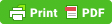## Related posts:

• Determine vector components
• Mass and weight – problems and solutions
• Particles in the one-dimensional equilibrium – application of the Newton’s first law problems and solutions
• Angular displacement and linear displacement – problems and solutions
• Mechanical equivalent of heat – problems and solutions
• Optical instrument eyeglasses – problems and solutions
• Work-mechanical energy principle – problems and solutions
• Perfectly elastic collisions in one dimension – problems and solutions
• Electric flux – problems and solutions
• Kinetic theory of gases – problems and solutions
• Transverse and longitudinal waves – problems and solutions
• Step-up and step-down transformer – problems and solutions
• Doppler effect – problems and solutions
• Free fall motion – problems and solutions
• Thermodynamics – problems and solutions
• Radial acceleration – problems and solutions
• Magnetic force between two parallel wires – problems and solutions
• Wheels connected by belts – problems and solutions
• The energy produced in the fusion reaction – problems and solutions
• Impulse equation#### IMAGES

1. Projectile Motion Problem Set #1 walkthrough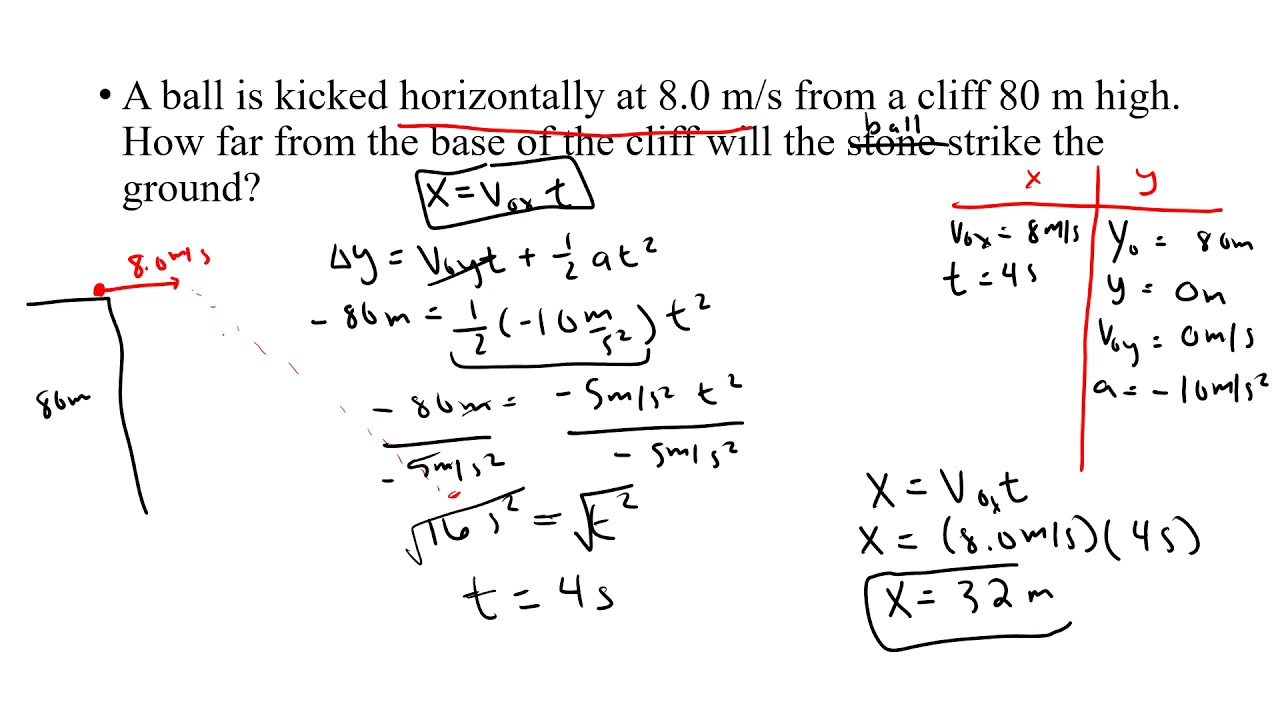2. PPT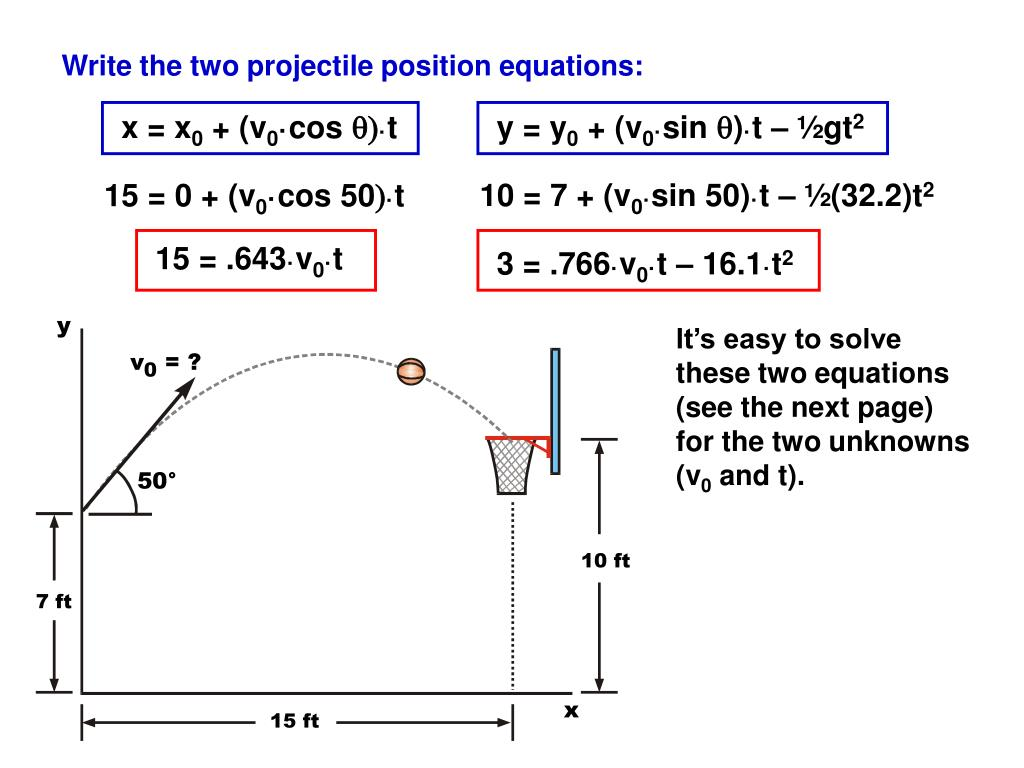3. Projectile Motion II4. Projectile Motion II5. (PDF) Projectile Motion-problems6. Projectile Motion: Practice Problems & Solutions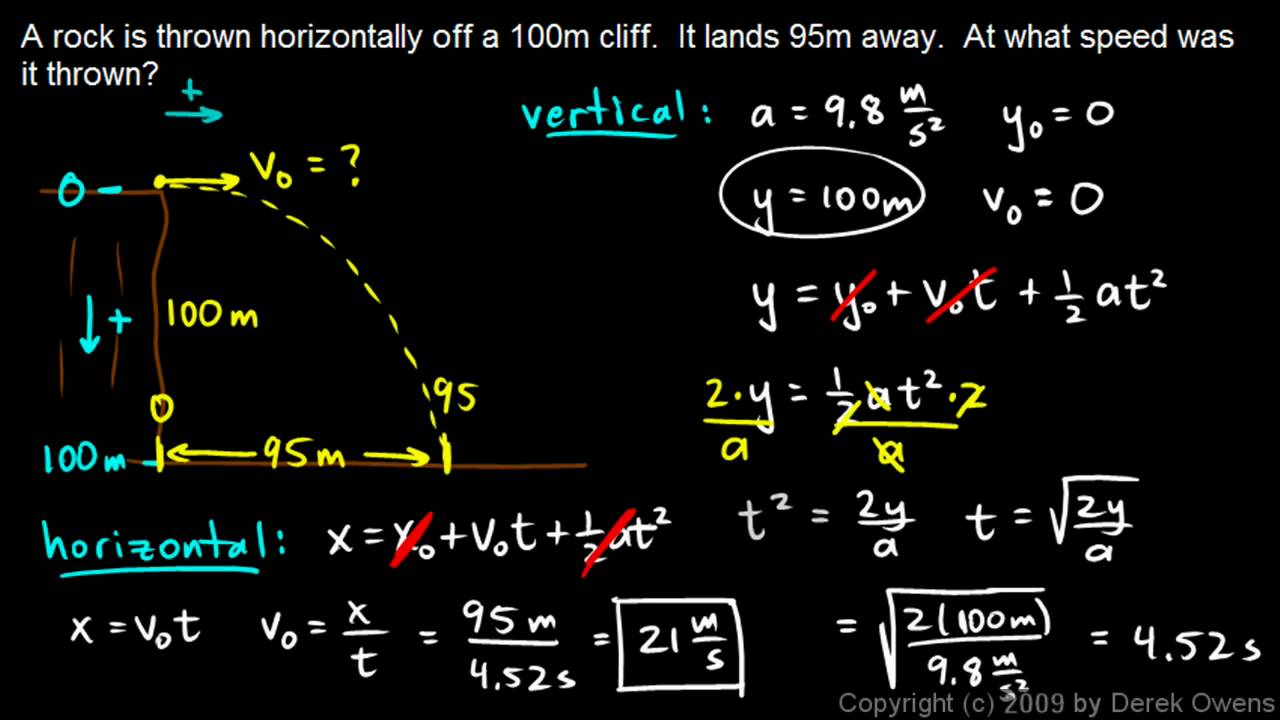#### VIDEO

1. Projectile motion problem

2. Projectile Motion Problem Physics-Find Distance given Horizontal Velocity

3. Projectile Motion Problem Solving (5 Examples & Strategies)

4. LEARN PHYSICS// PROJECTILE MOTION//PROBLEM SOLVING METHOD

5. Projectile Motion Problems || CBSE || ICSE || CLASS 11 || JEE/NEET || PHYSICS

6. Projectile Motion:Cliff Problems

1. PDF Projectile Motion: Practice Problems with Solutions Physexams

Solution: This is a projectile motion problem, a type of motion in which, without air resis- tance, we have ax = 0 and ay = −g. The kinematic equations for projectile are: = (v0 cos α) t z v0x = − gt2 + (v0 sin α) t + y0 zv0y vx = v0 cos α zv0x vy = v0 sin α −gt zv0y

2. PDF Projectile problems

Projectile model When an object is projected with velocity V at an angle of to the horizontal and is then assumed to move freely under gravity in a vertical plane, its motion can be modelled by the following equations: (x, y) V Horizontal motion x V cos t y Vertical motion y V sin t 1 gt 2

3. Projectile Motion Practice Problems for AP Physics

There is also a pdf for worksheets with answers. Overview of projectile motion: Any motion having the following conditions is called a projectile motion. (i) Follows a parabolic path (trajectory). (ii) Moves under the influence of gravity.

4. PDF SHOW YOUR WORK. 1. 2. 3. 4.

Projectile Motion Problem Worksheet Answer Key SHOW YOUR WORK. Acceleration due to gravity: a = 9.81 m/s2 ) A ball is dropped from 4 meters above the ground. If it begins at rest, how long does it take to hit the ground? Use Equation 2 from above. Replace xf and x0 with yf and y0, respectively, to show that the ball moves vertically.

5. 3.3: Projectile Motion

Projectile motion is a form of motion where an object moves in a bilaterally symmetrical, parabolic path. The path that the object follows is called its trajectory. Projectile motion only occurs when there is one force applied at the beginning of the trajectory, after which the only interference is from gravity.

6. PDF Projectile motion

10 Horizontal Projectile Problem #2 You are at the top of a 3.5 m high stair case. A friend at the bottom of the stairs forgot her pencil and asks you for one. You notice that she is 11 Projectiles Launched at an Angle When projectiles are launched at an angle, they are given an initial horizontal and vertical velocity. The horizontal 12 14

7. PDF Projectile and Circular Motion

Projectile Motion Kinematic Equations for 2-D: Must be able to identify variables in these equations! Projectile Motion: special case where 𝑎 = 0 and 𝑎 = Uniform Circular Motion Speed is constant Direction is changing Acceleration toward center 𝑎𝑐=𝑣2/ , and 𝑎 =0 = 0+𝑣0 + 1 2 𝑎 2

8. PDF Teacher Toolkit Topic: Objectives

This simulation with accompanying problem set would be ideal for a digital lab on projectile motion. The worksheet was crafted to help learners stay focused on specific questions limited in scope to launch angle and initial speed. It's a solid way to introduce learners to projectile motion without overwhelming them with too many variables. 3.

9. PDF MOTION OF A PROJECTILE

MOTION OF A PROJECTILE (Section 12.6) Projectile motion can be treated as two rectilinear motions, one in the horizontal direction experiencing zero acceleration and the other in the vertical direction experiencing constant acceleration (i.e., gravity). For illustration, consider the two balls on the left. The red ball falls from rest, whereas the

10. PDF TOPIC 1.4: PROJECTILE MOTION

S4P-1-17 Calculate the horizontal and vertical components with respect to velocity and position of a projectile at various points along its path. S4P-1-18 Solve problems for projectiles launched horizontally and at various angles to the horizontal to calculate maximum height, range, and overall time of flight of the projectile.

11. PROJECTILE MOTION PRACTICE QUESTIONS (WITH ANSWERS)

The diagram shows a projectile being launched at a c Determine the speed of the projectile 1.0 s velocity of 1.0 km s-1 at an angle of 30° to the after it is launched. horizontal. Assume that g = 9.8 m s-2 and that air resistance is negligible.

12. PDF Chapter 5 Projectiles 5 PROJECTILES

• know how to use the model to investigate real life projectile problems. 5.0 Introduction What do tennis and basket balls have in common with kangaroos? The ball or body is in motion through the air, the only forces acting on it being its weight and the resistance to its motion due to the air. A motion like this is called a projectile motion and

13. Horizontally Launched Projectile Problems

The two types of problems are: Problem Type 1: A projectile is launched with an initial horizontal velocity from an elevated position and follows a parabolic path to the ground. Predictable unknowns include the initial speed of the projectile, the initial height of the projectile, the time of flight, and the horizontal distance of the projectile.

14. Projectile Problems with Solutions and Explanations

Problem 1 An object is launched at a velocity of 20 m/s in a direction making an angle of 25° upward with the horizontal. a) What is the maximum height reached by the object? b) What is the total flight time (between launch and touching the ground) of the object? c) What is the horizontal range (maximum x above ground) of the object?

15. Vectors and Projectiles Problem Sets

Includes 6 problems. Problem Set VP2 - Relative Motion 2. Determine the velocity of an object with respect to an observer if the object is moving along a medium that moves relative to the observer. Includes 5 problems. Problem Set VP3 - Resolving Vectors into Components 1.

16. 4.4: Projectile Motion

Legal. Accessibility Statement For more information contact us at [email protected]. Projectile motion is the motion of an object subject only to the acceleration of gravity, where the acceleration is constant, as near the surface of Earth. To solve projectile motion problems, we ….

17. 5.3 Projectile Motion

Projectile motion is the motion of an object thrown (projected) into the air when, after the initial force that launches the object, air resistance is negligible and the only other force that object experiences is the force of gravity. The object is called a projectile, and its path is called its trajectory.

18. PDF Solutions of Projectile Motion Exercise-1 Part

A-1. T1 = 2usin(90 - ) ; T2 = sin 2 sin(90- = tan ) {or T1 : T2 = tan : 1 2 sin 2 A-2. H = , 2g 2 sin 2 H1 = , 2 sin 2 (90- ) H2 = 2 H 1 H sin cos A-3. Horizontal Vertical height given R = 2 sin2 So 2×2 sin tan = 4 4. R same 2 = 90 - 2usin = g

19. Projectile Motion Problem Solving

Step 1: Calculate the time required for the car to freefall from a height of 72 m. h = viyt + 1 2gt2 but since viy = 0, the equation simplifies to h = 1 2gt2 rearranging for the unknown variable, t, yields. t = √2h g = √ 2(72 m) 10.0 m / s2 = 3.79 s. Step 2: Solve for initial velocity: vix = d t = 22 m 3.79 s = 5.80 m / s.

20. Projectile Motion Worksheet Solutions-Odds PDF

Download now. of 4. PROJECTILE MOTION WORKSHEET 1A ball is kicked horizontally at 8.0 m/s from a cliff 80m high. How far from the base of the cliff will the stone strike the ground? 2. How long will it take a shell fired from a cliff at an initial velocity of 800 mis at an angle 30° 'below the horizontal to reach the ground 150m below? 3.

21. Vectors and Projectiles Problem Sets

Problem 1: Coach Sweeney walks 26 yards to the north along the sideline, pauses, and walks 12 yards back to the south. a. Determine the distance which Coach moved. b. Determine Coach's resultant displacement. Audio Guided Solution Show Answer Problem 2: a.

22. Projectile motion

Solution : The initial velocity at the horizontal direction (x axis) : vox = vo cos 60o = (20) (0.5) = 10 m/s The initial velocity at the vertical direction (y axis) : voy = vo sin 60o = (20) (0.5√3) = 10√3 m/s The time interval to reach the maximum height, calculated using this equation : vty = voy + g t

23. Solving a projectile motion problem by thinking like a physicist

Coop-Problem-Solving-Guide.pdf)  Serway R A and Jewett J W 2014 Physics for Scientists and Engineers 9th edn (Boston, MA: Brooks) p 86  Mungan C E 2017 Optimizing the launch of a projectile to hit a target Phys. Teach. 55 528-9  Rizcallah J A 2018 Projectile motion without calculus Phys. Educ. 23 045002 Launch Target g x y y' a' x' y